# ICSE Class 8 Maths Selina Solutions for Chapter 18 Constructions

The chapter constructions come under the unit Geometry which discusses about drawing of shapes, angles or lines accurately. These constructions are done only by using a compass, straightedge and a pencil. In this chapter, you will learn pure form of geometric constructions of quadrilaterals where no numbers will be involved. For the convenience of the students, we have provided ICSE Class 8 Maths Selina Solutions Chapter 18 Constructions prepared by our in-house subject experts in a simple and understandable manner. By going through these solutions students will get an idea on how to solve the questions.

These Selina Solutions will also help them while preparing for their final exam. Students can access the pdf of ICSE Class 8 Maths Selina Solutions of Chapter 18 Constructions from the link provided below. Solve these questions before the exams to clear your doubts related to the chapter.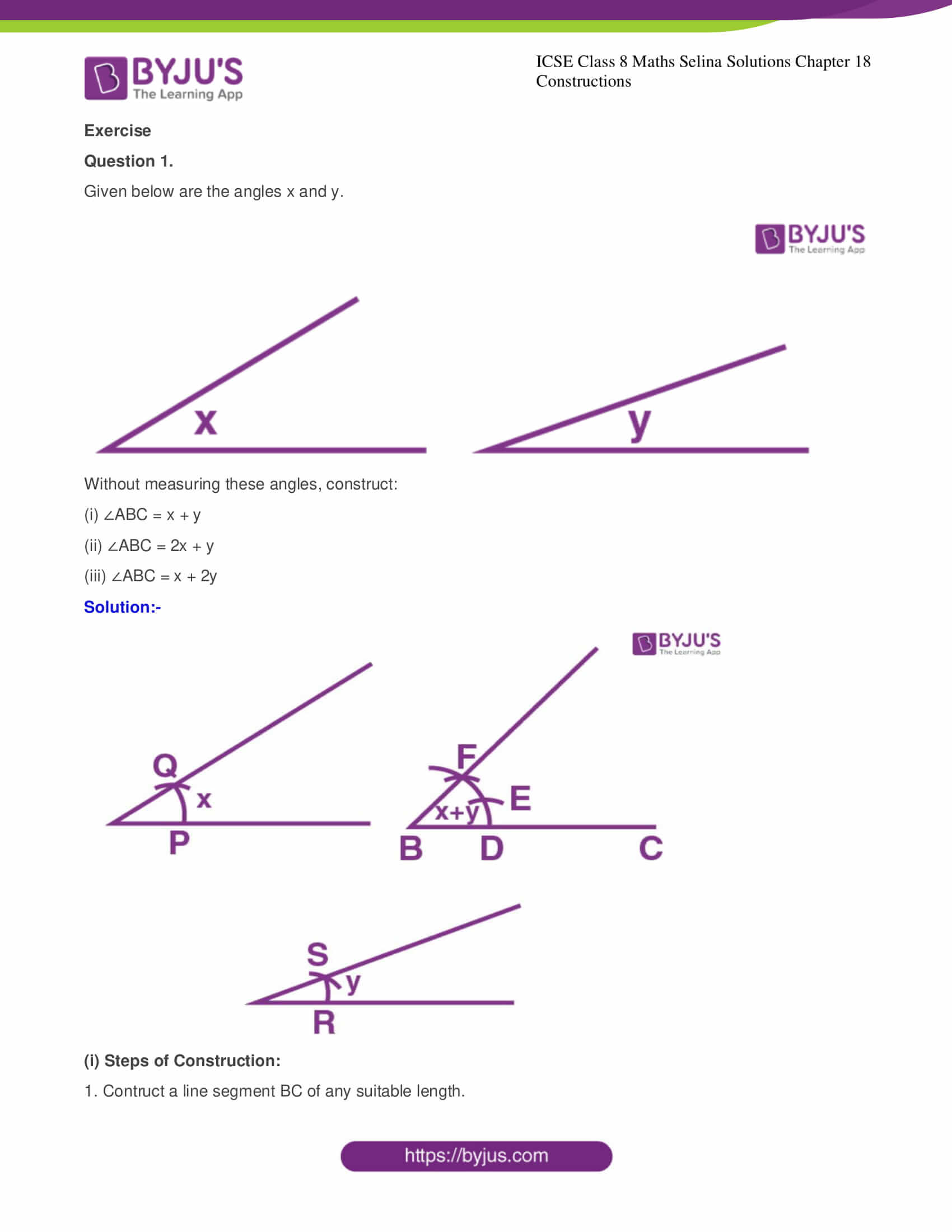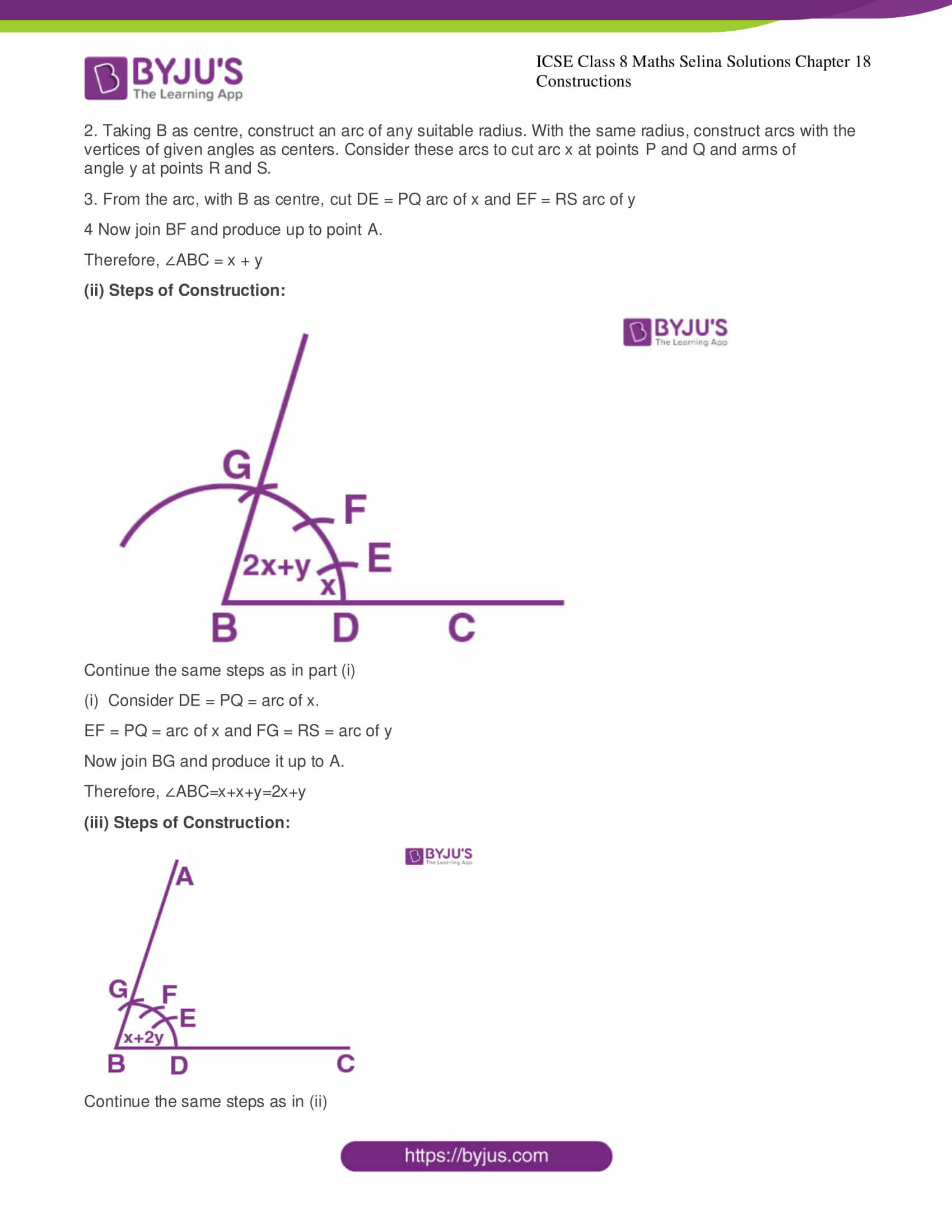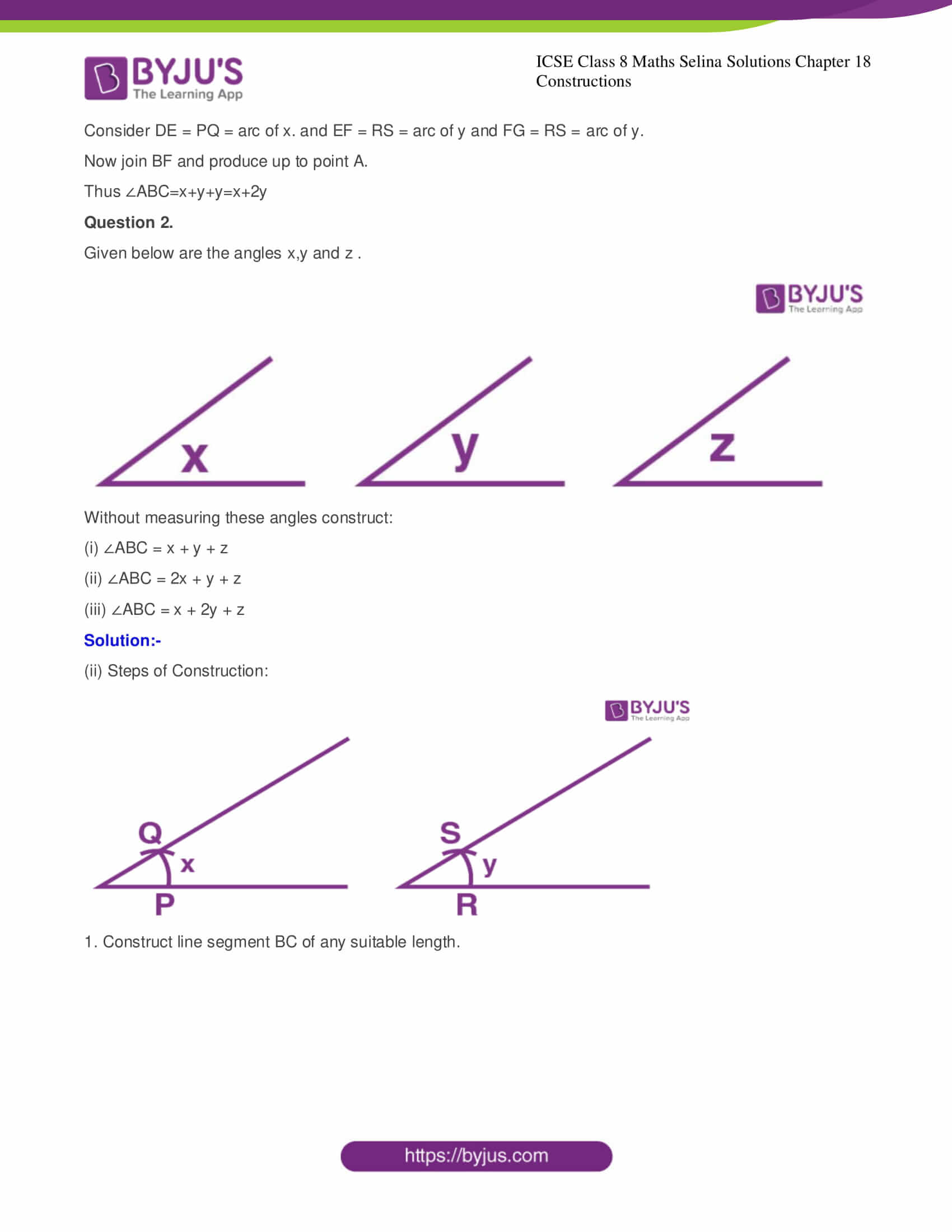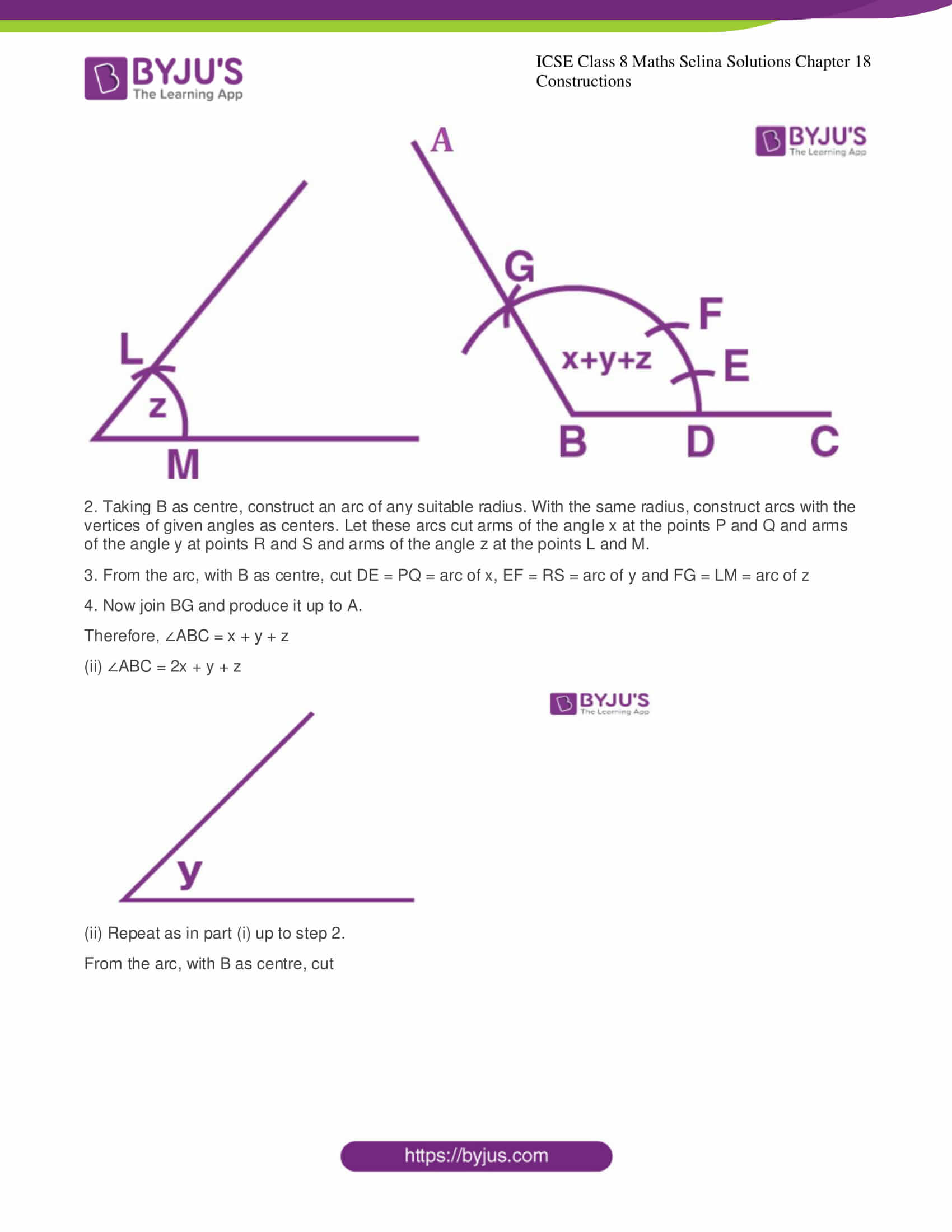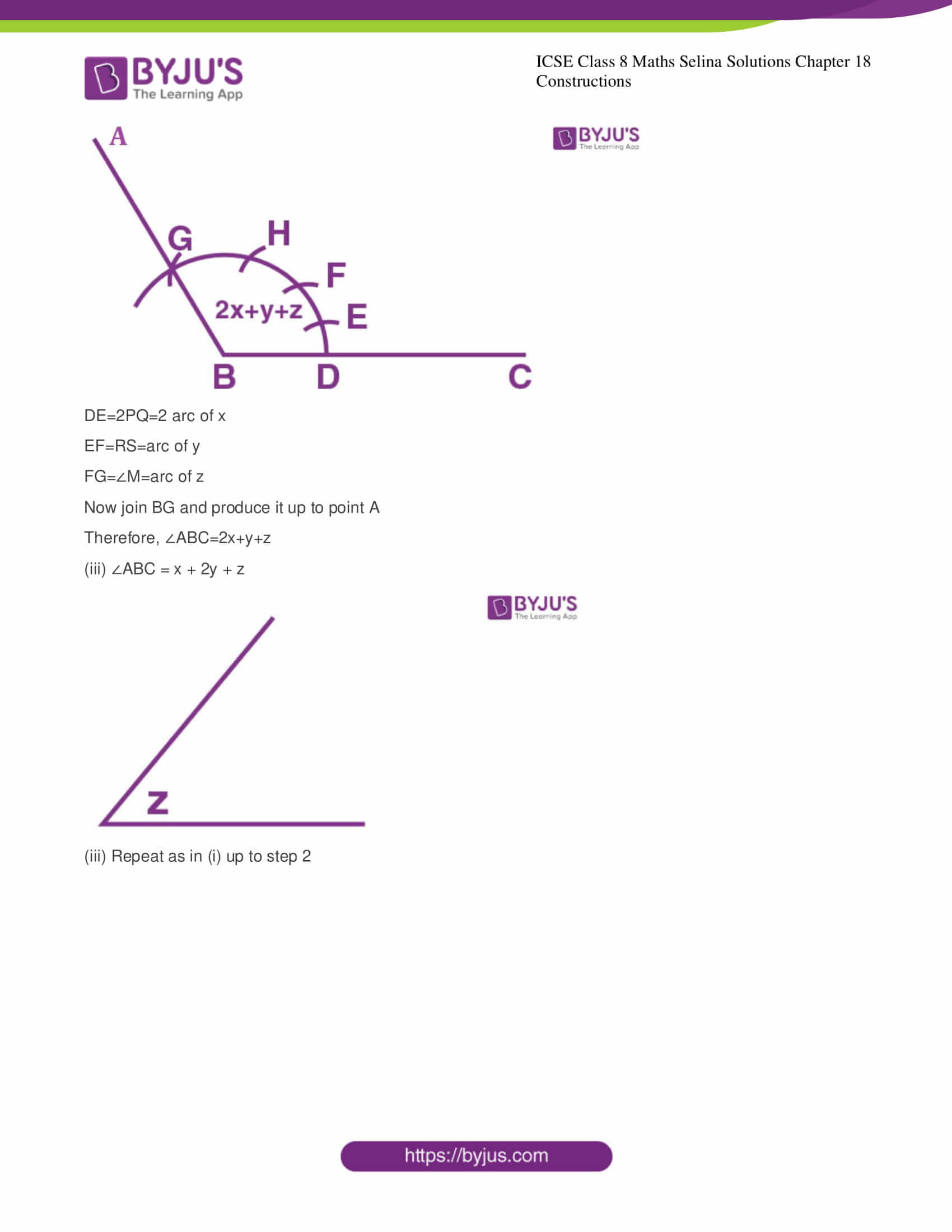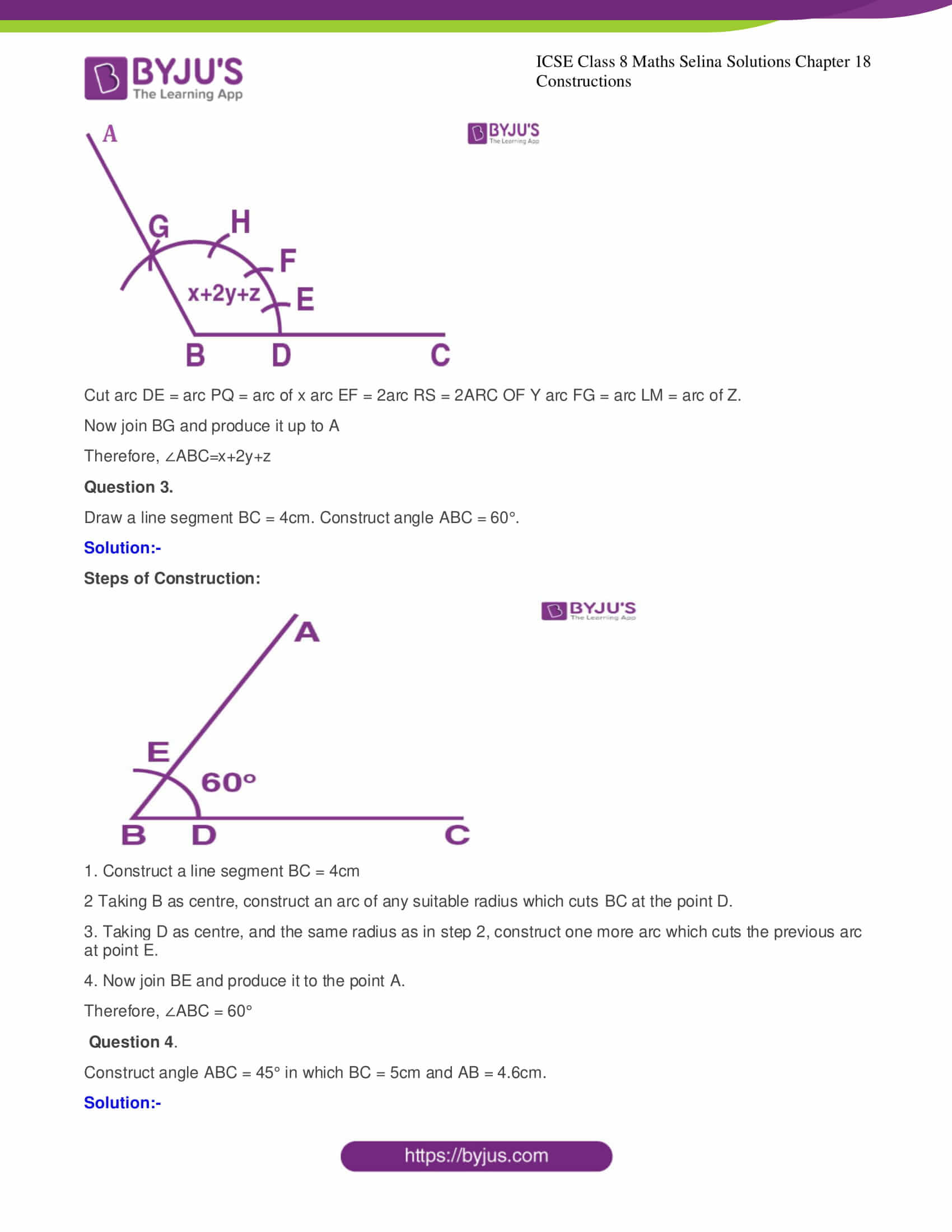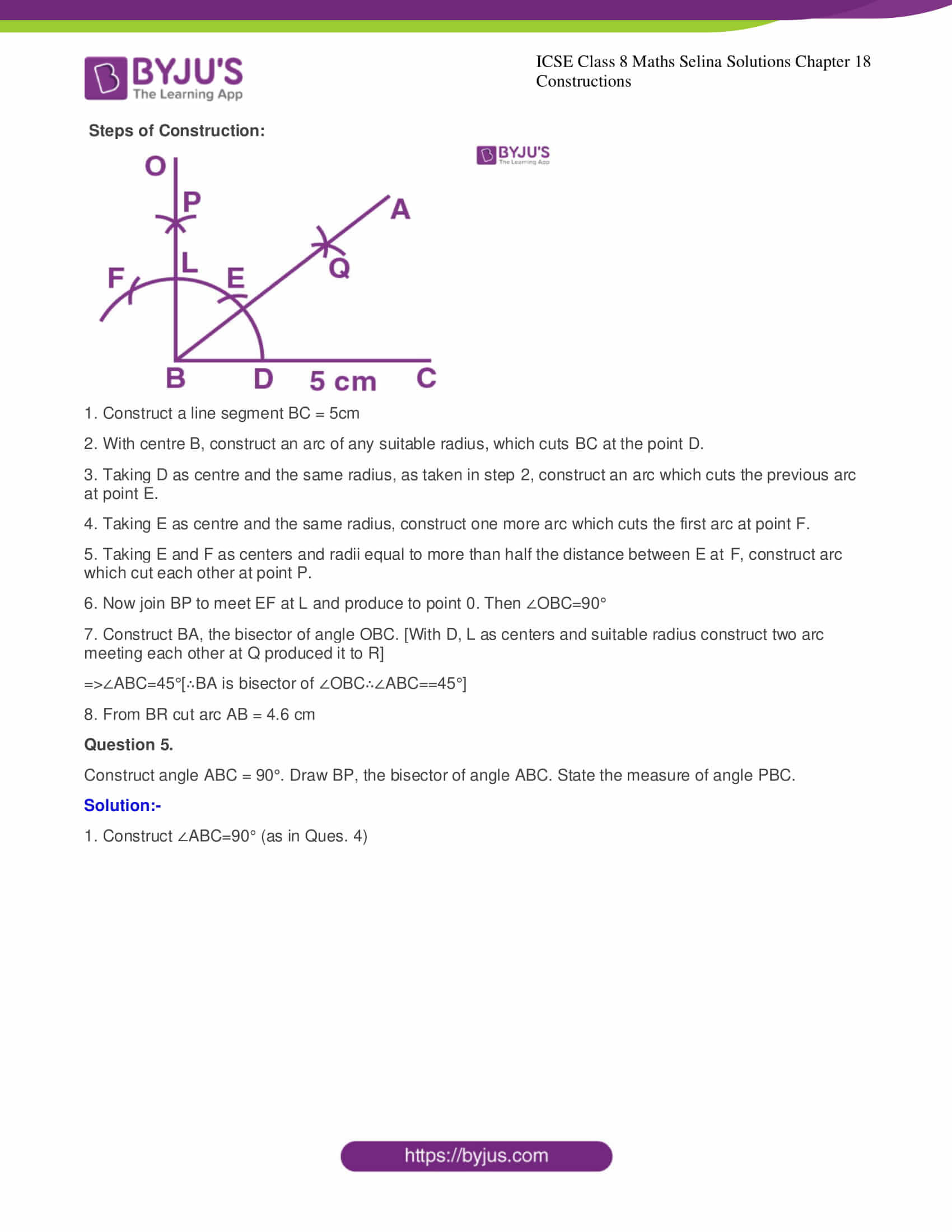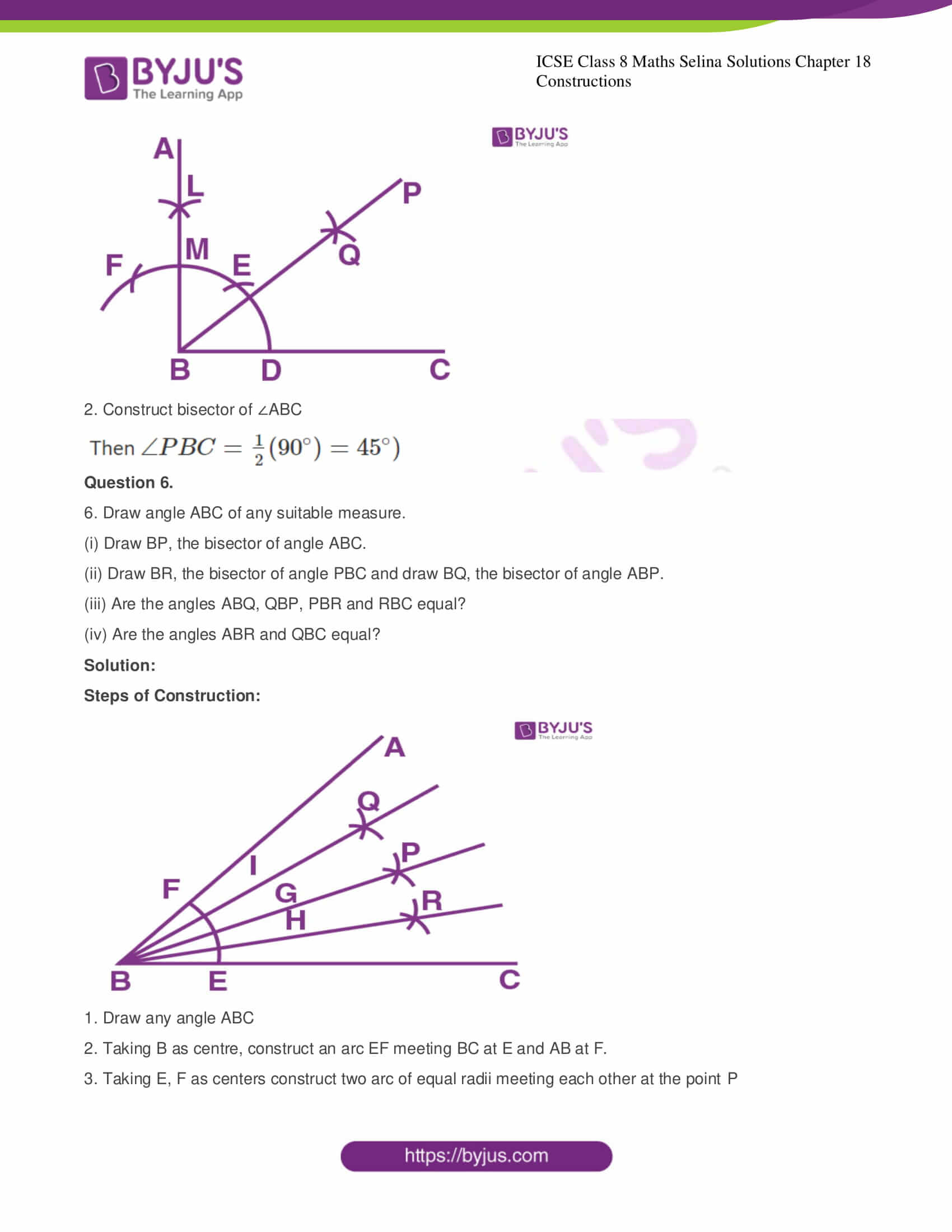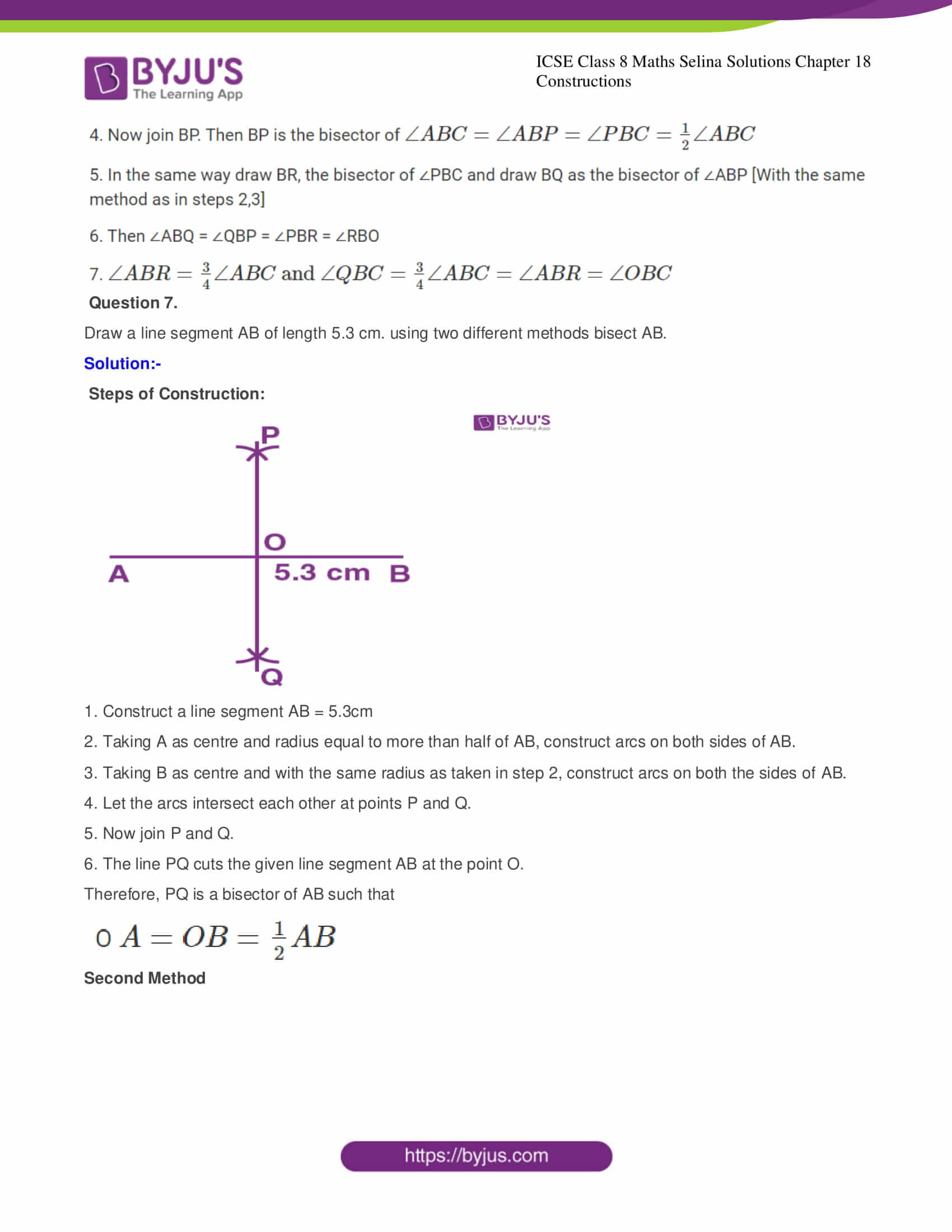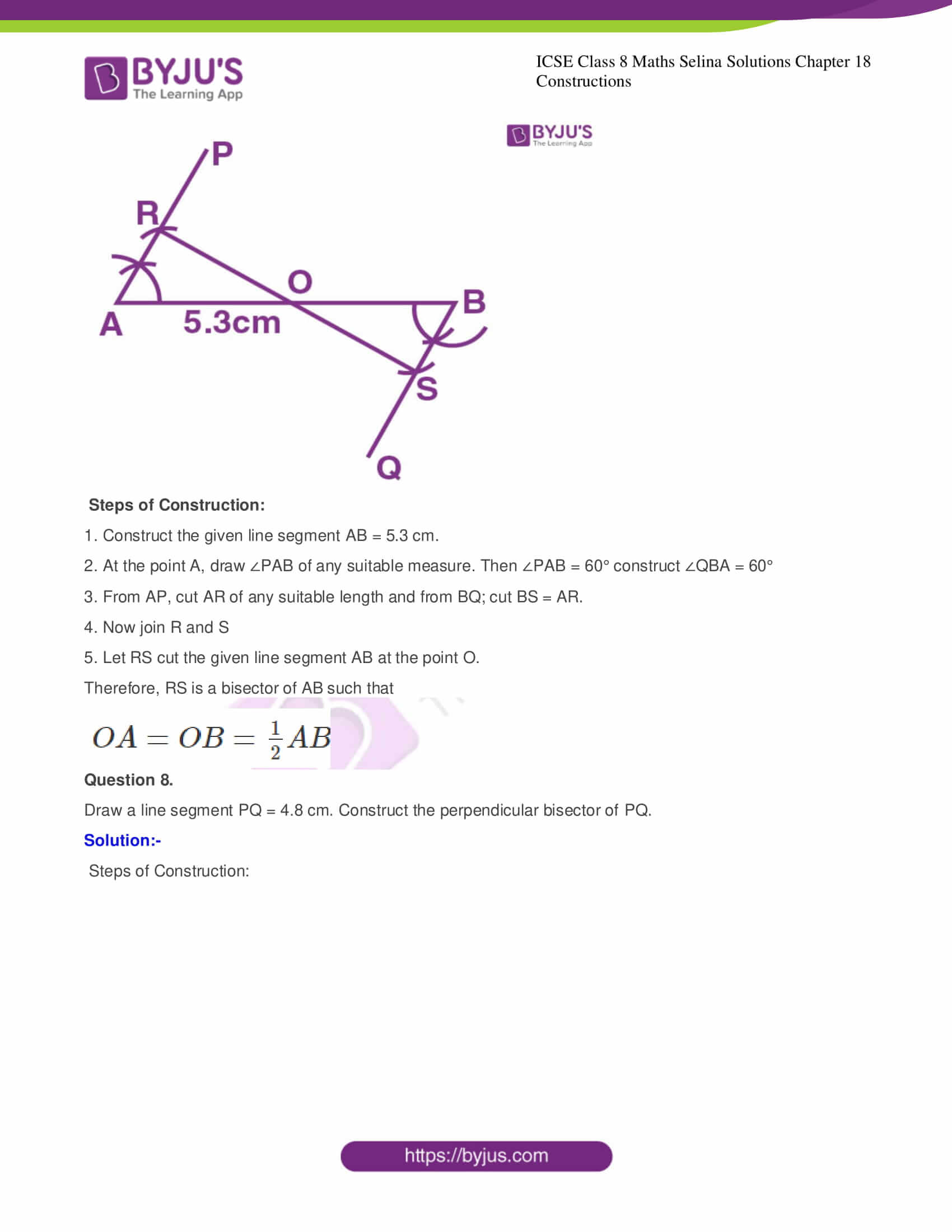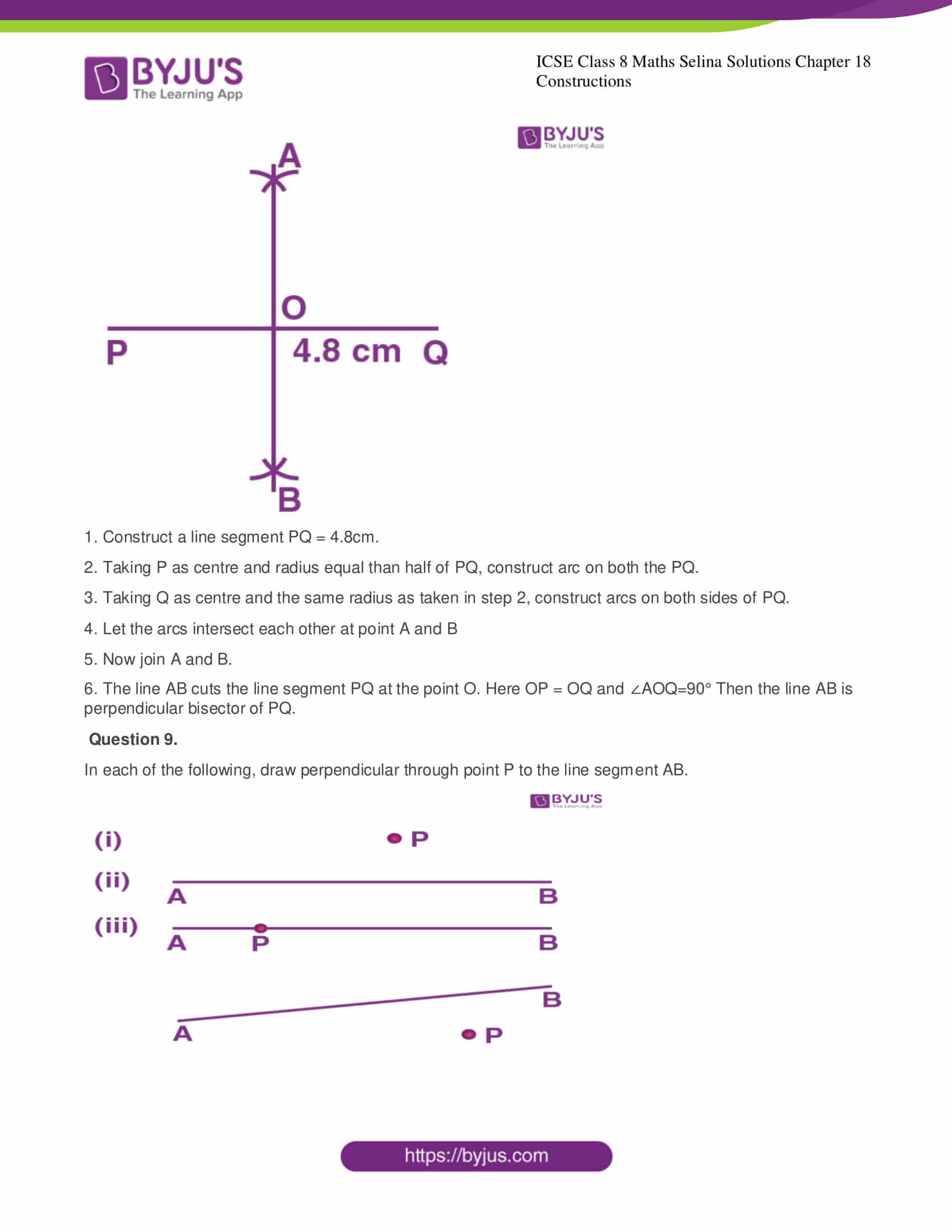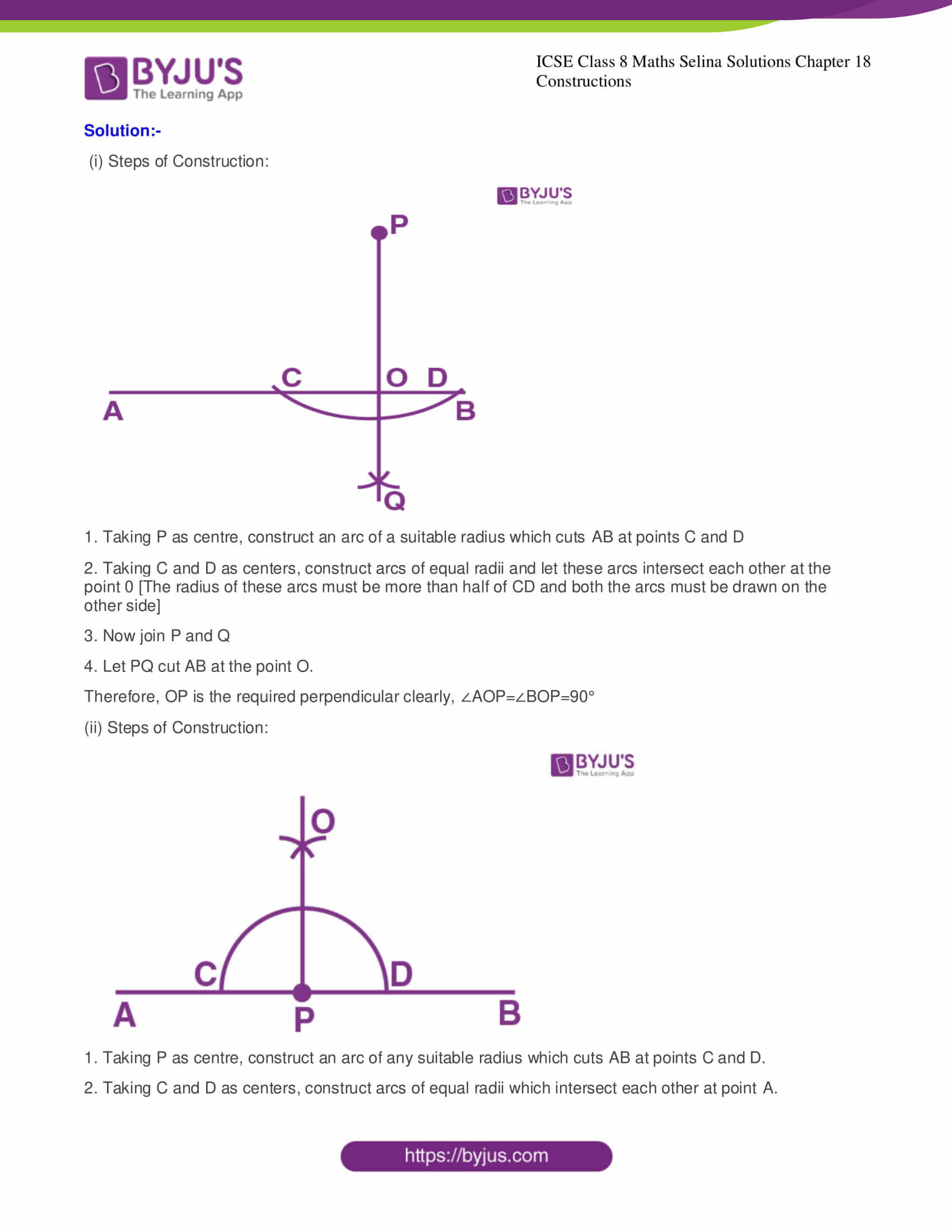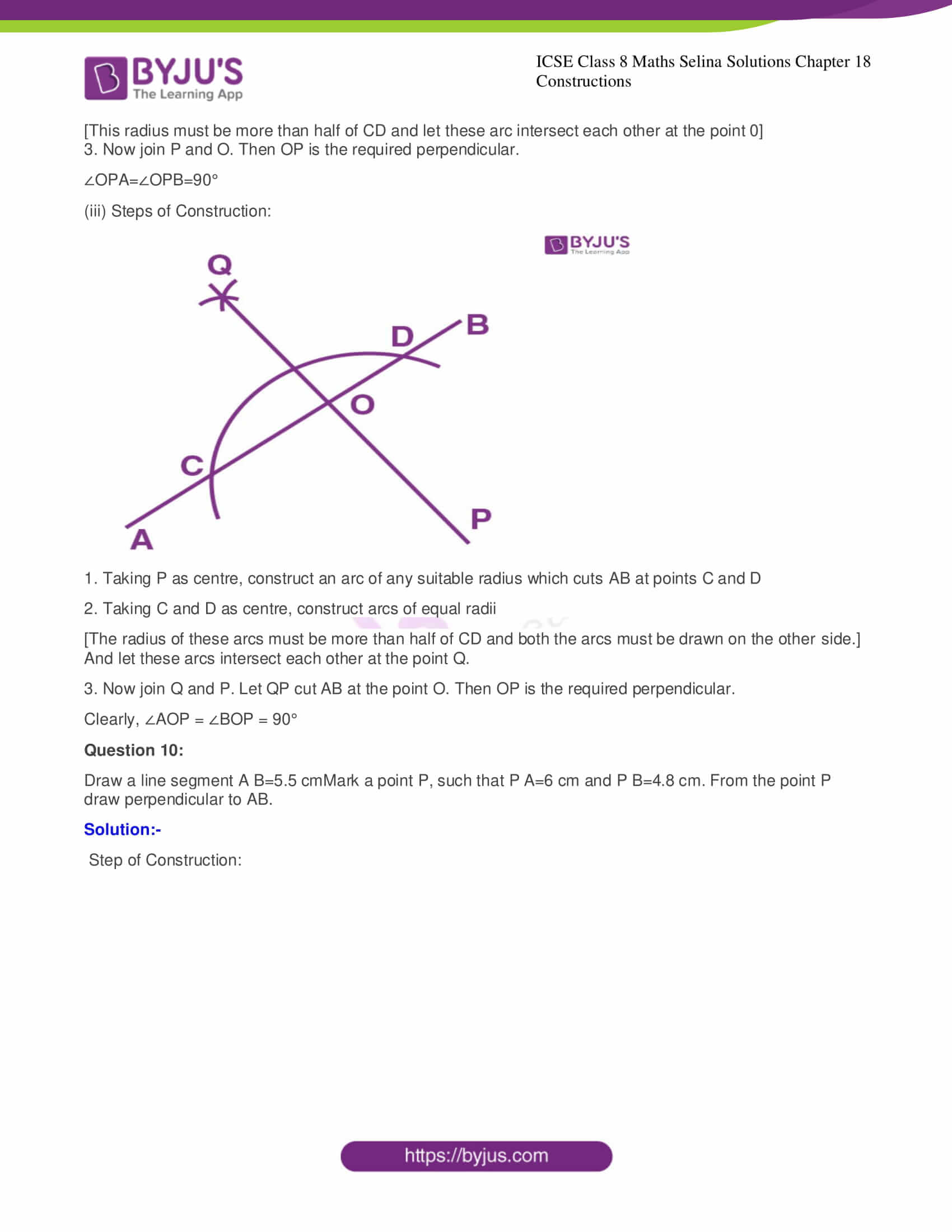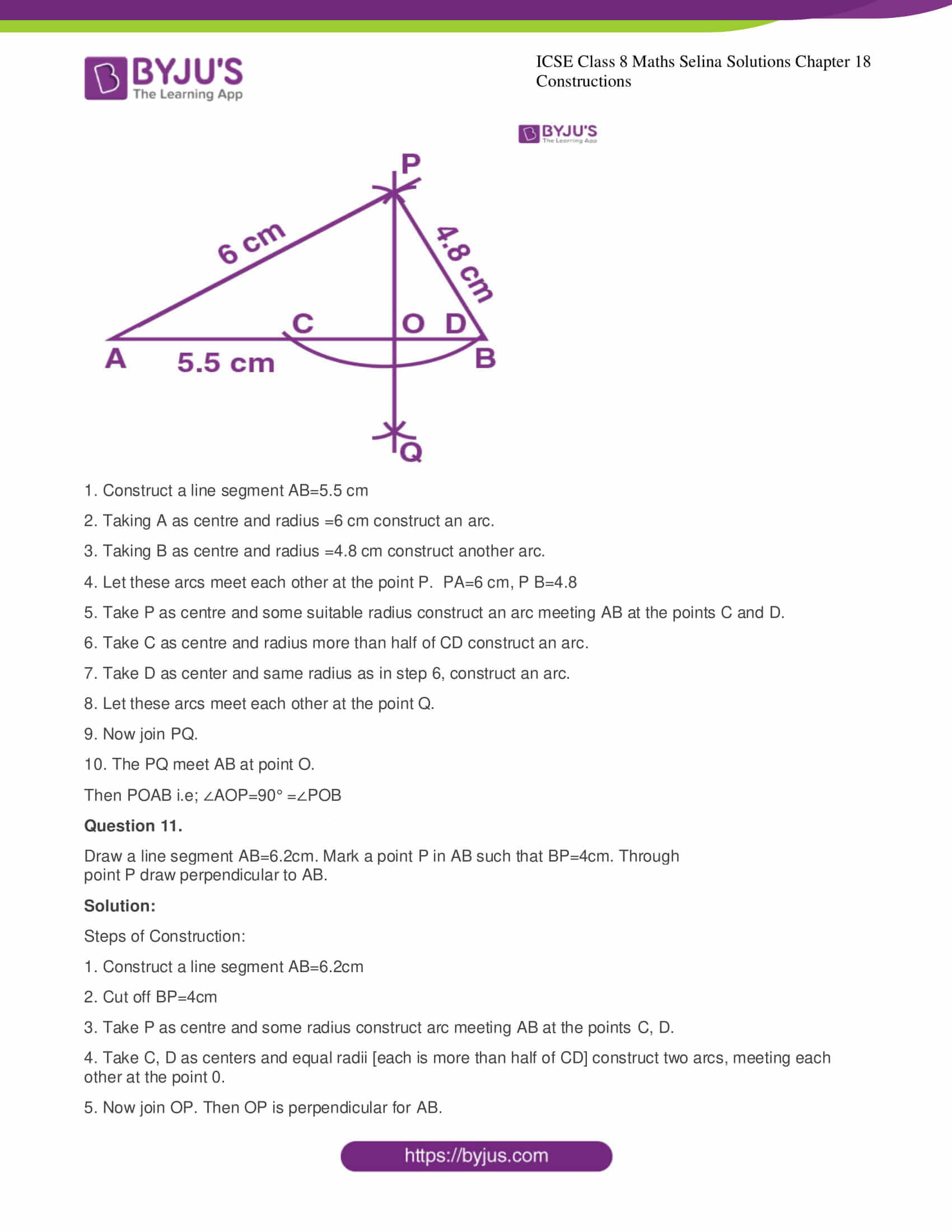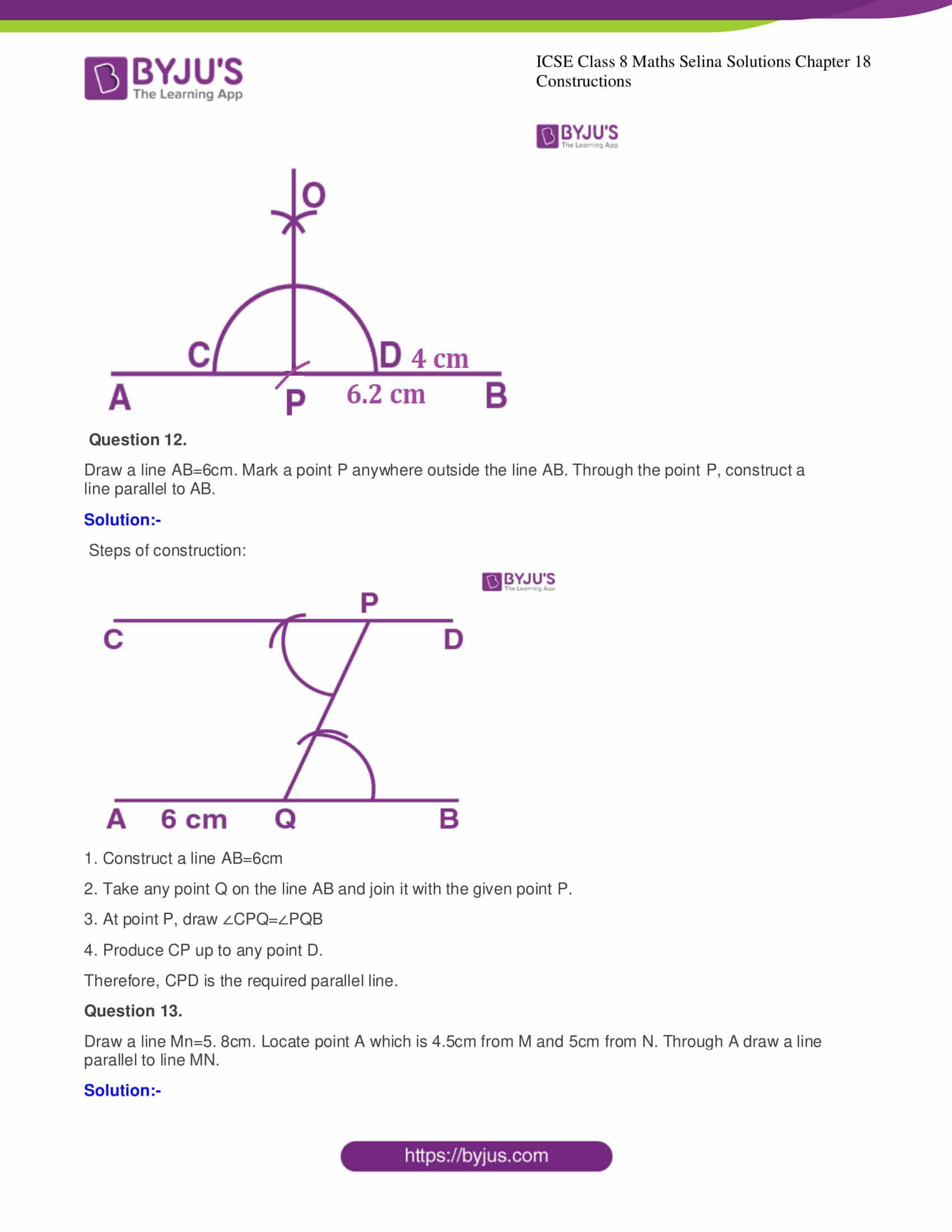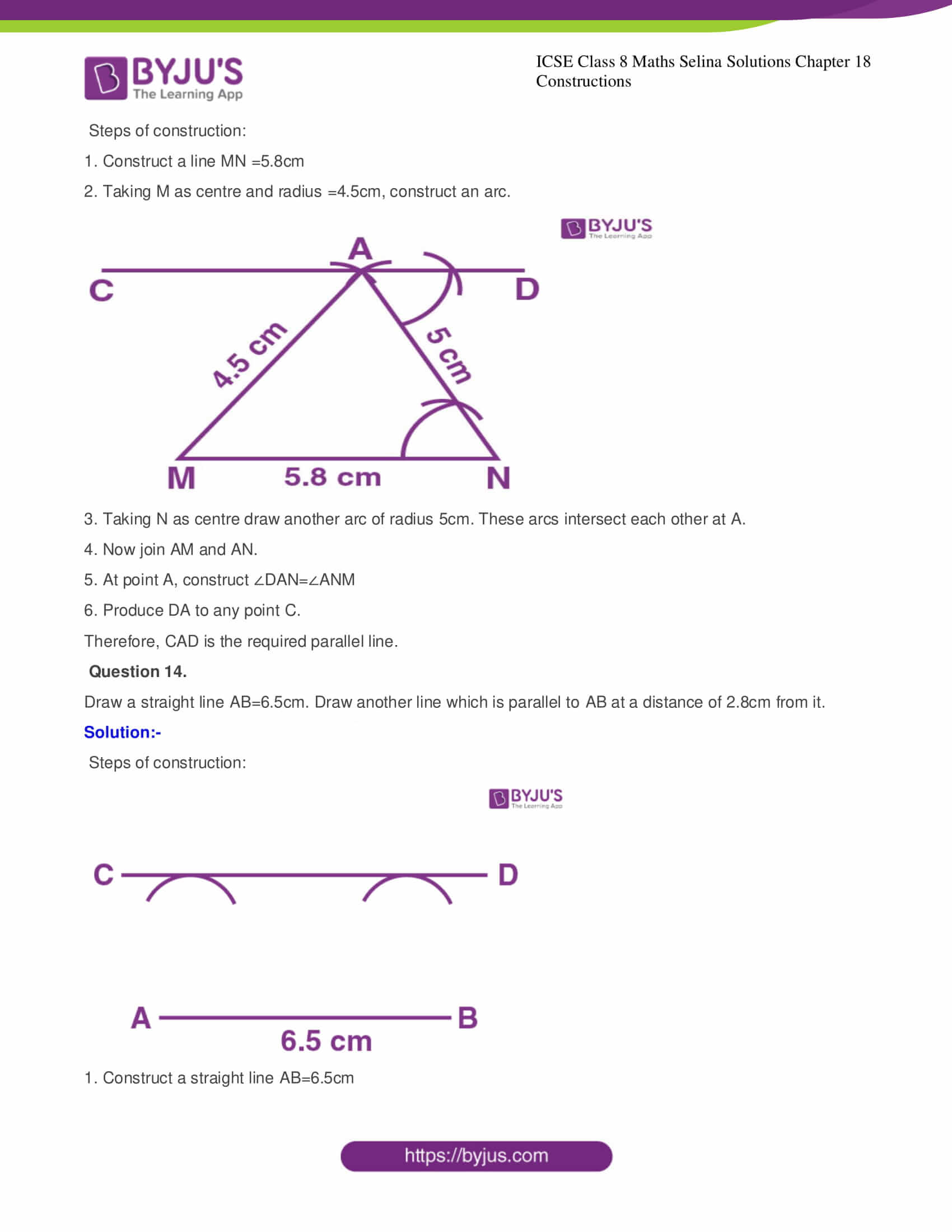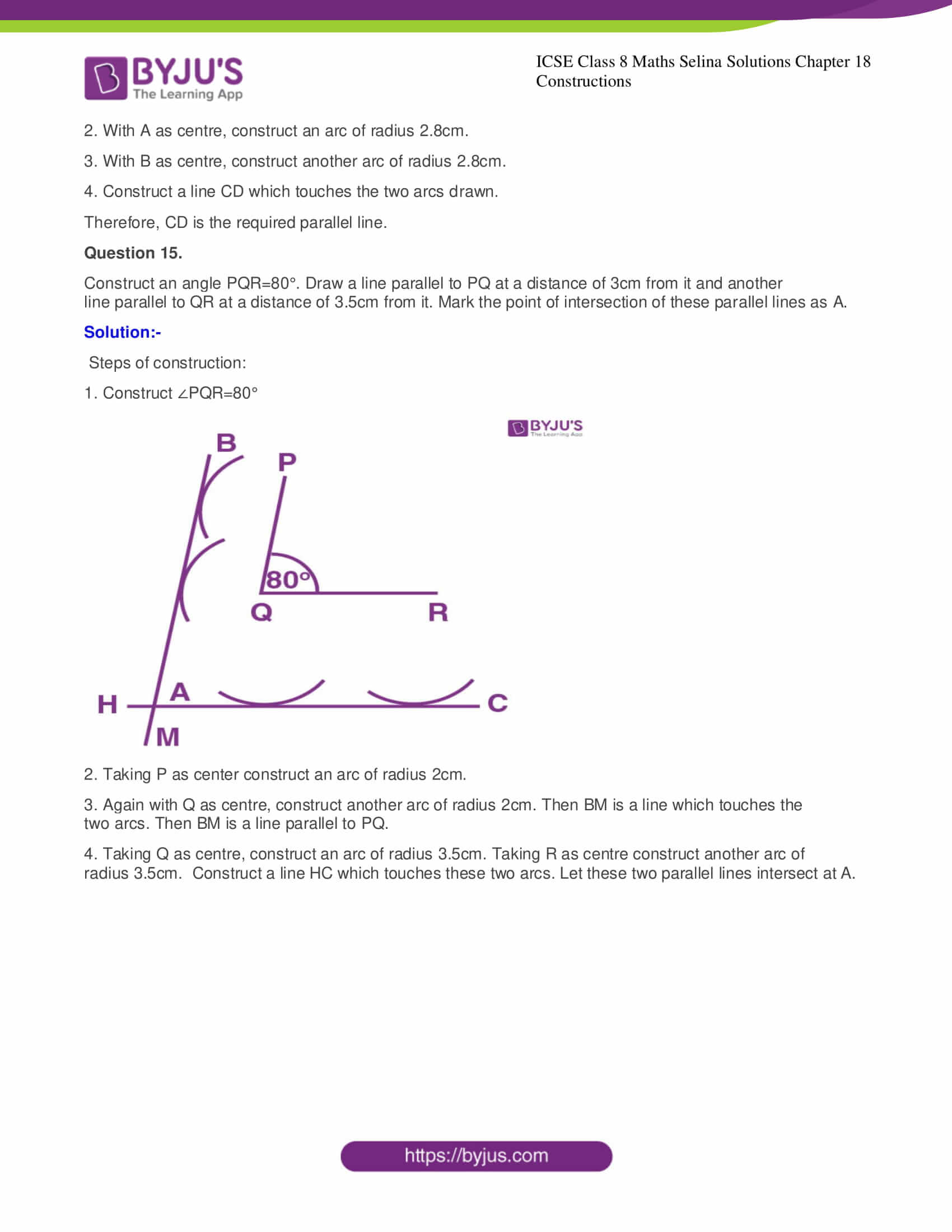The exercise mentioned in Chapter 18 Constructions have a total of 15 questions and the detailed solutions of these questions are provided below.

### CHAPTER 18 – CONSTRUCTIONS

Exercise

Question 1.

Given below are the angles x and y.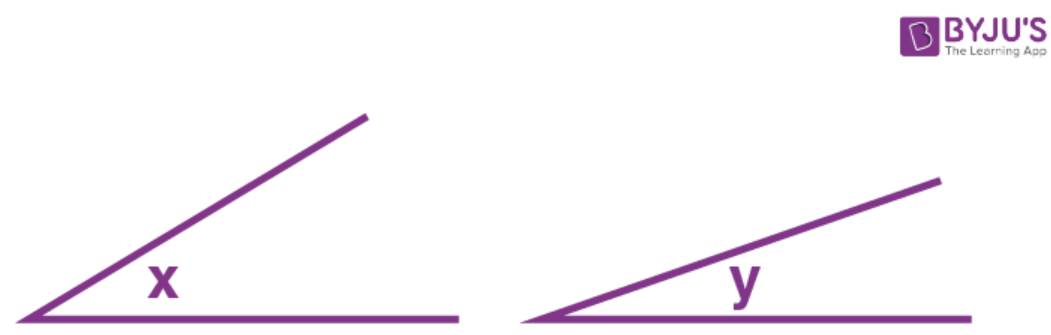Without measuring these angles, construct:

(i) ∠ABC = x + y

(ii) ∠ABC = 2x + y

(iii) ∠ABC = x + 2y

Solution:-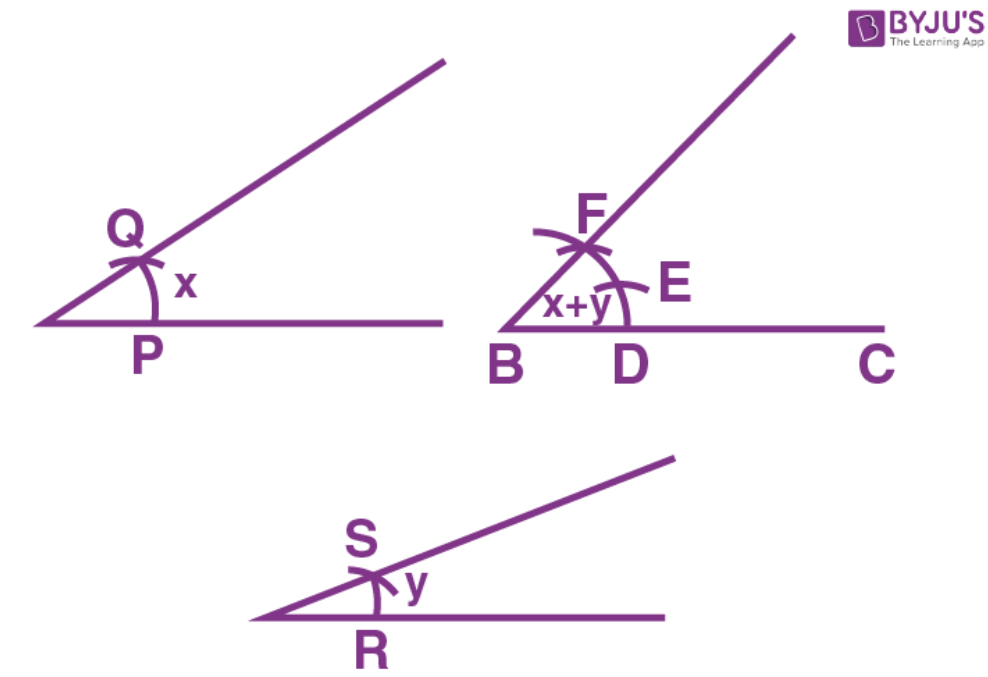(i) Steps of Construction:

1. Contruct a line segment BC of any suitable length.

2. Taking B as centre, construct an arc of any suitable radius. With the same radius, construct arcs with the vertices of given angles as centers. Consider these arcs to cut arc x at points P and Q and arms of angle y at points R and S.

3. From the arc, with B as centre, cut DE = PQ arc of x and EF = RS arc of y

4 Now join BF and produce up to point A.

Therefore, ∠ABC = x + y

(ii) Steps of Construction: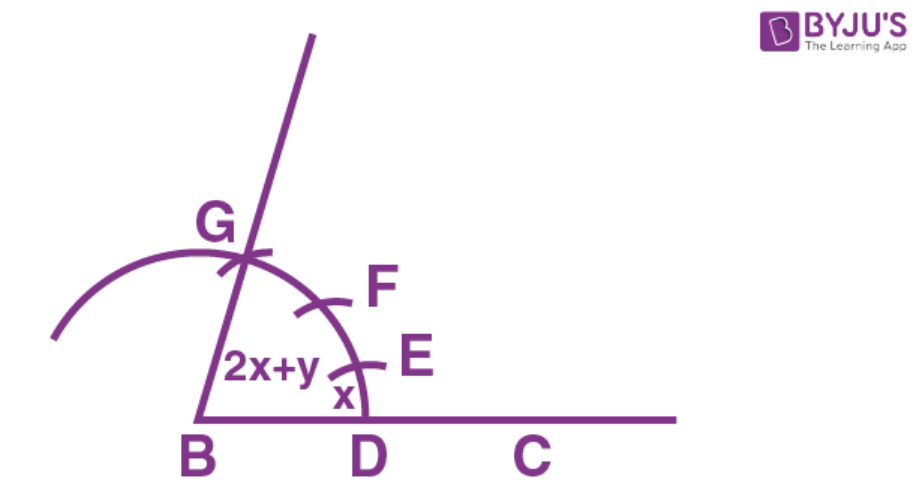Continue the same steps as in part (i)

(i)  Consider DE = PQ = arc of x.

EF = PQ = arc of x and FG = RS = arc of y

Now join BG and produce it up to A.

Therefore, ∠ABC=x+x+y=2x+y

(iii) Steps of Construction: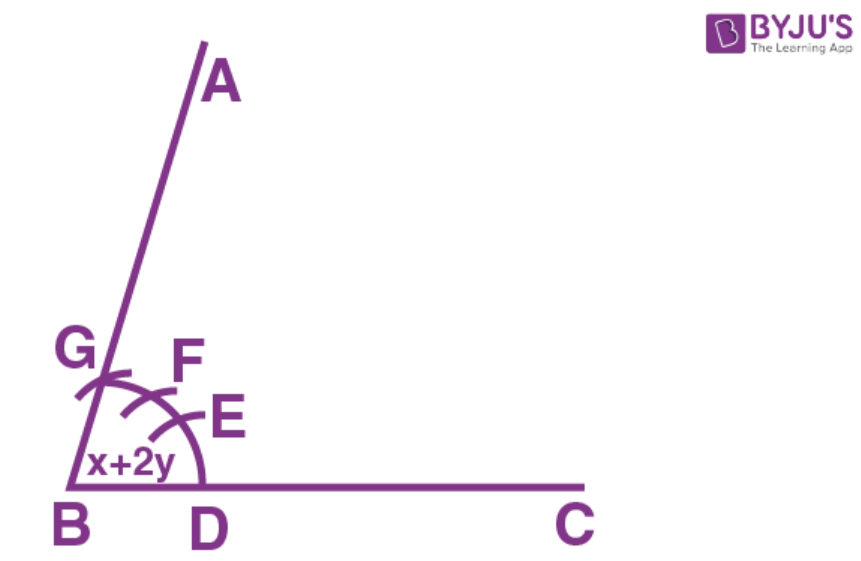Continue the same steps as in (ii)

Consider DE = PQ = arc of x. and EF = RS = arc of y and FG = RS = arc of y.

Now join BF and produce up to point A.

Thus ∠ABC=x+y+y=x+2y

Question 2.

Given below are the angles x,y and z .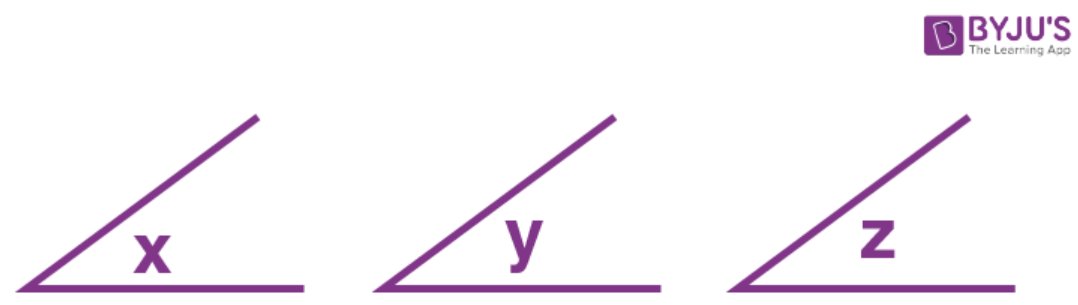Without measuring these angles construct:

(i) ∠ABC = x + y + z

(ii) ∠ABC = 2x + y + z

(iii) ∠ABC = x + 2y + z

Solution:-

(ii) Steps of Construction: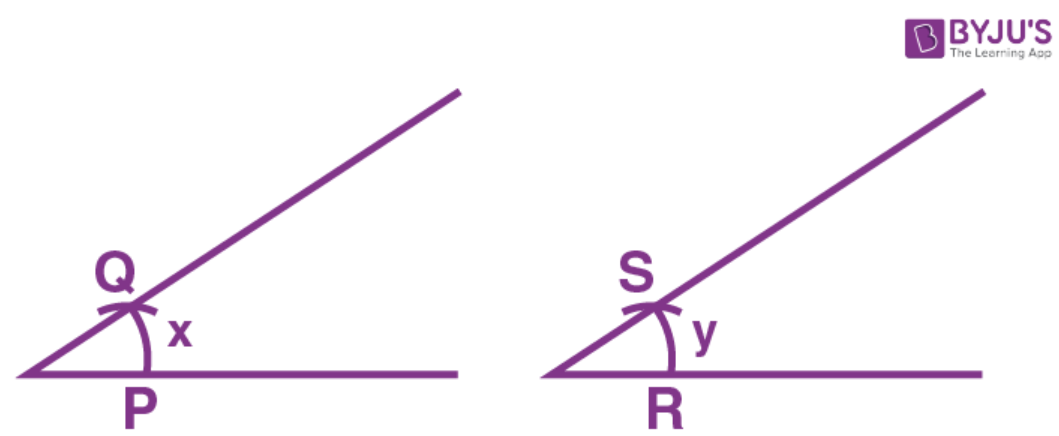1. Construct line segment BC of any suitable length.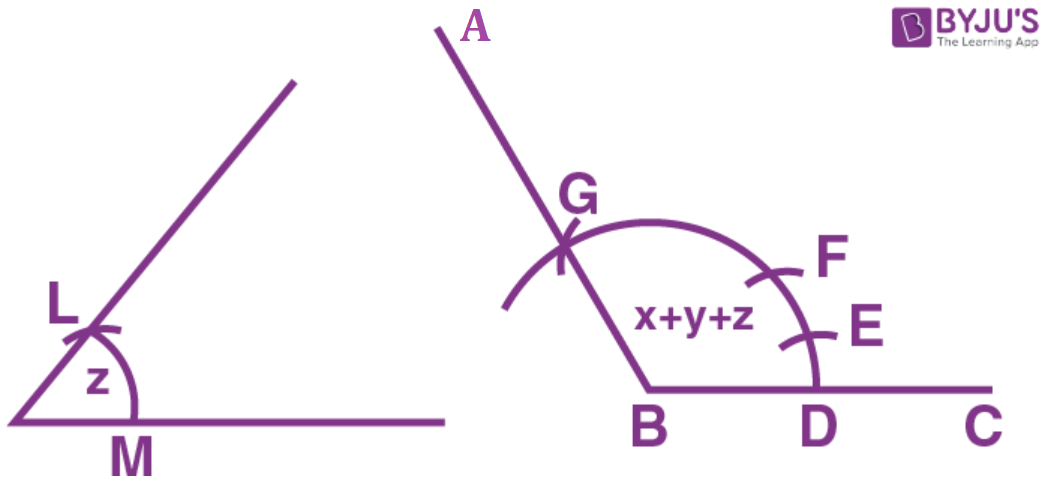2. Taking B as centre, construct an arc of any suitable radius. With the same radius, construct arcs with the vertices of given angles as centers. Let these arcs cut arms of the angle x at the points P and Q and arms of the angle y at points R and S and arms of the angle z at the points L and M.

3. From the arc, with B as centre, cut DE = PQ = arc of x, EF = RS = arc of y and FG = LM = arc of z

4. Now join BG and produce it up to A.

Therefore, ∠ABC = x + y + z

(ii) ∠ABC = 2x + y + z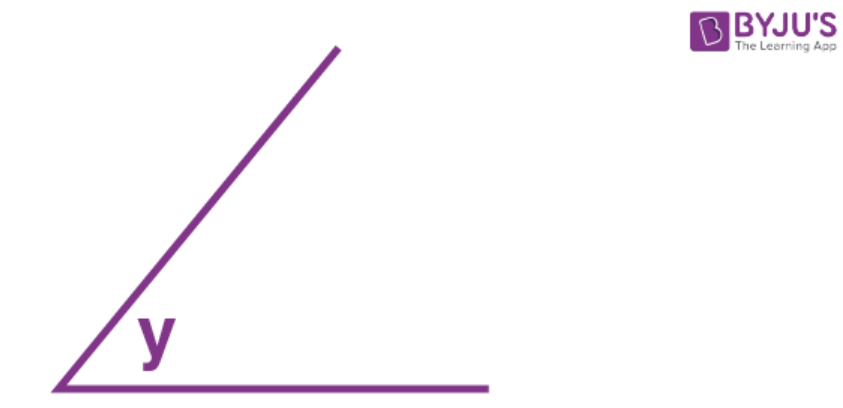(ii) Repeat as in part (i) up to step 2.

From the arc, with B as centre, cut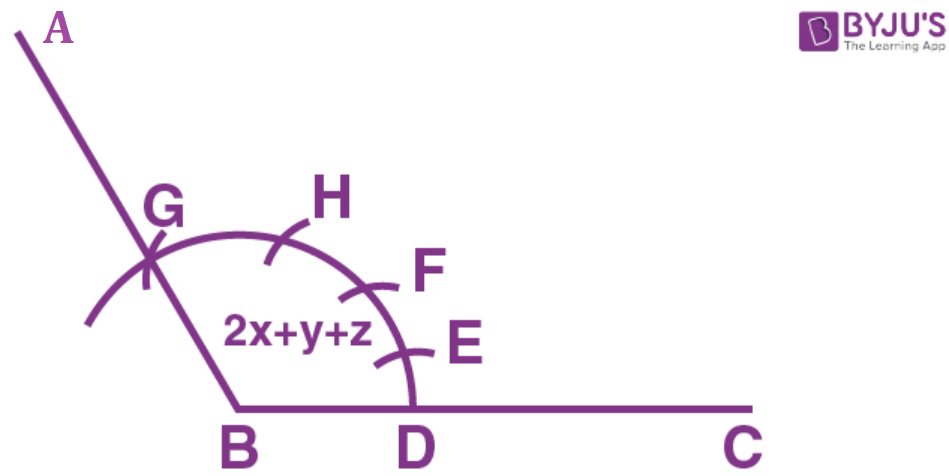DE=2PQ=2 arc of x

EF=RS=arc of y

FG=∠M=arc of z

Now join BG and produce it up to point A

Therefore, ∠ABC=2x+y+z

(iii) ∠ABC = x + 2y + z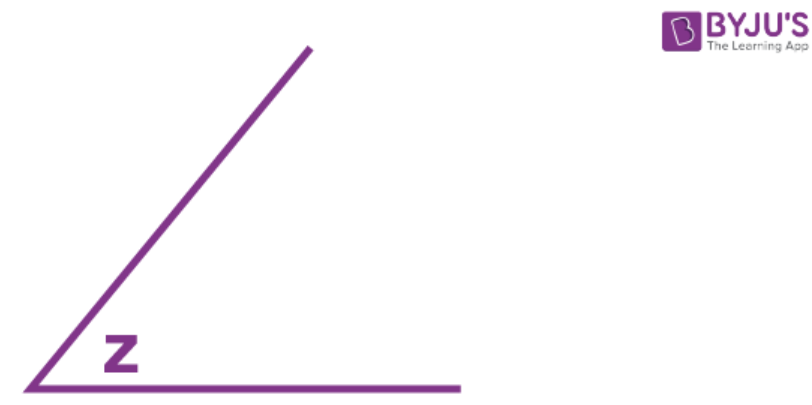(iii) Repeat as in (i) up to step 2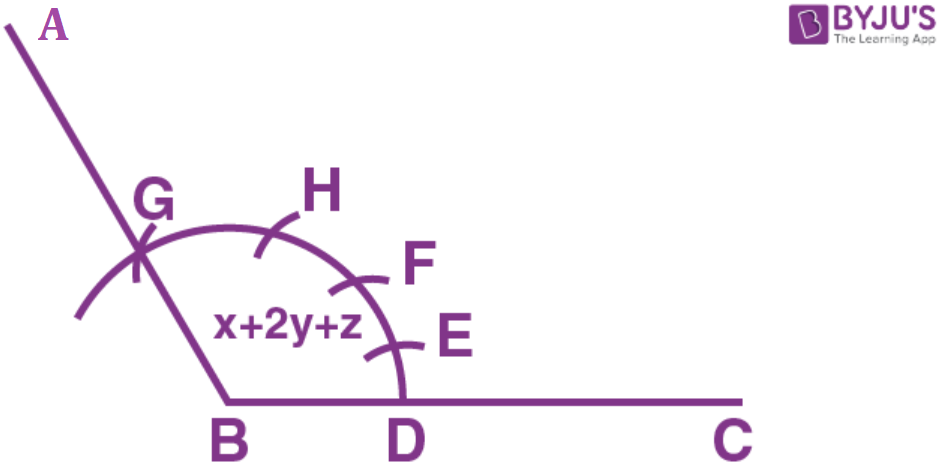Cut arc DE = arc PQ = arc of x arc EF = 2arc RS = 2ARC OF Y arc FG = arc LM = arc of Z.

Now join BG and produce it up to A

Therefore, ∠ABC=x+2y+z

Question 3.

Draw a line segment BC = 4cm. Construct angle ABC = 60°.

Solution:-

Steps of Construction: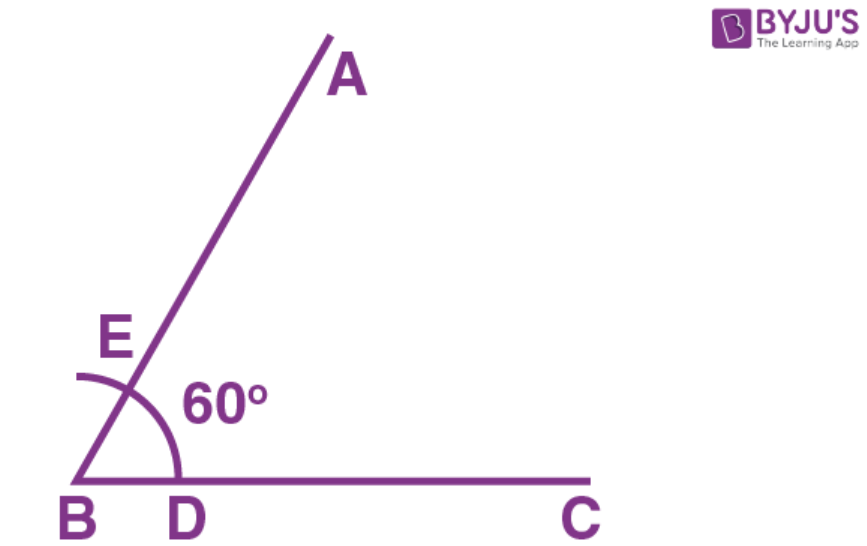1. Construct a line segment BC = 4cm

2 Taking B as centre, construct an arc of any suitable radius which cuts BC at the point D.

3. Taking D as centre, and the same radius as in step 2, construct one more arc which cuts the previous arc at point E.

4. Now join BE and produce it to the point A.

Therefore, ∠ABC = 60°

Question 4.

Construct angle ABC = 45° in which BC = 5cm and AB = 4.6cm.

Solution:-

Steps of Construction: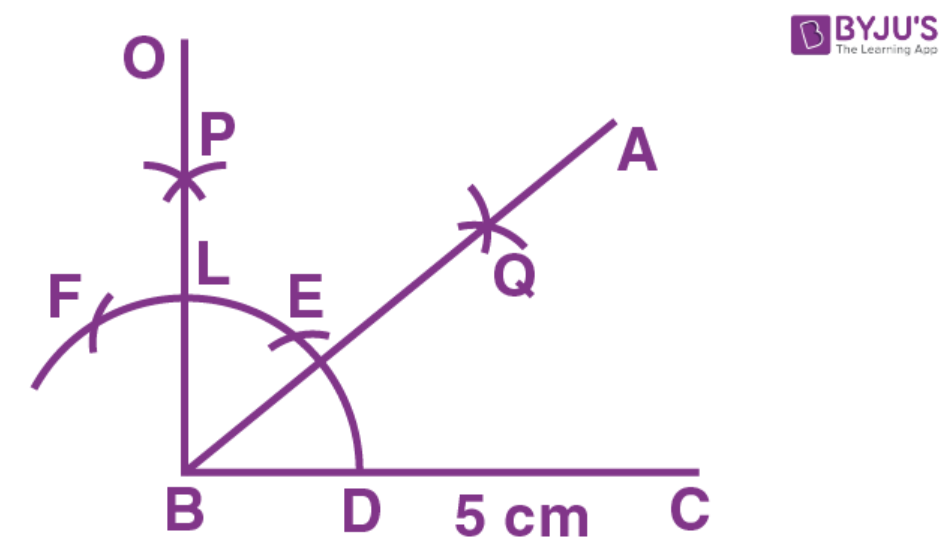1. Construct a line segment BC = 5cm

2. With centre B, construct an arc of any suitable radius, which cuts BC at the point D.

3. Taking D as centre and the same radius, as taken in step 2, construct an arc which cuts the previous arc at point E.

4. Taking E as centre and the same radius, construct one more arc which cuts the first arc at point F.

5. Taking E and F as centers and radii equal to more than half the distance between E at F, construct arc which cut each other at point P.

6. Now join BP to meet EF at L and produce to point 0. Then ∠OBC=90°

7. Construct BA, the bisector of angle OBC. [With D, L as centers and suitable radius construct two arc meeting each other at Q produced it to R]

=>∠ABC=45°[∴BA is bisector of ∠OBC∴∠ABC==45°]

8. From BR cut arc AB = 4.6 cm

Question 5.

Construct angle ABC = 90°. Draw BP, the bisector of angle ABC. State the measure of angle PBC.

Solution:-

1. Construct ∠ABC=90° (as in Ques. 4)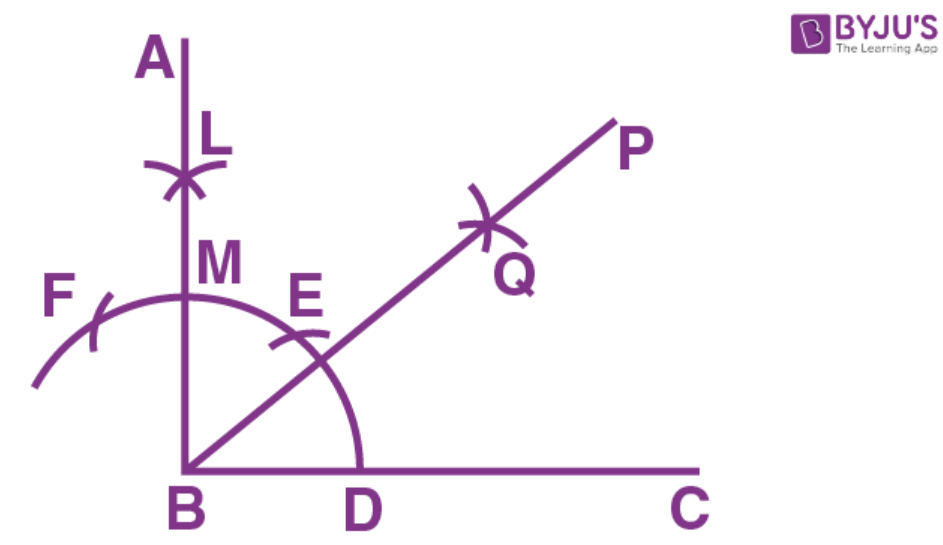2. Construct bisector of ∠ABC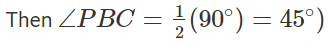Question 6.

6. Draw angle ABC of any suitable measure.

(i) Draw BP, the bisector of angle ABC.

(ii) Draw BR, the bisector of angle PBC and draw BQ, the bisector of angle ABP.

(iii) Are the angles ABQ, QBP, PBR and RBC equal?

(iv) Are the angles ABR and QBC equal?

Solution:

Steps of Construction: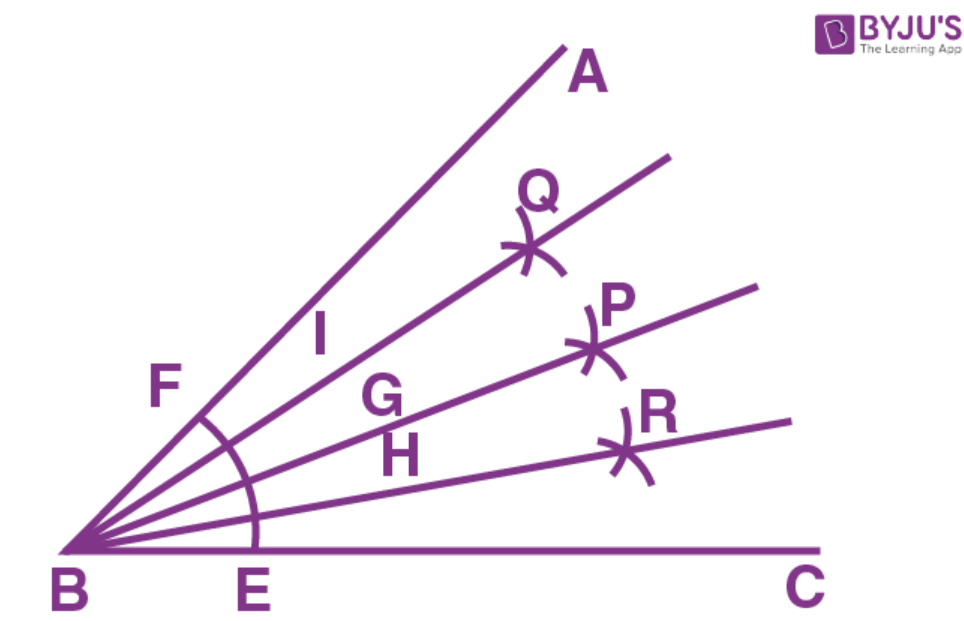1. Draw any angle ABC

2. Taking B as centre, construct an arc EF meeting BC at E and AB at F.

3. Taking E, F as centers construct two arc of equal radii meeting each other at the point P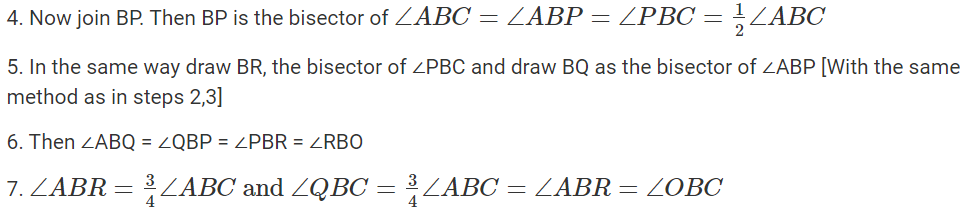Question 7.

Draw a line segment AB of length 5.3 cm. using two different methods bisect AB.

Solution:-

Steps of Construction: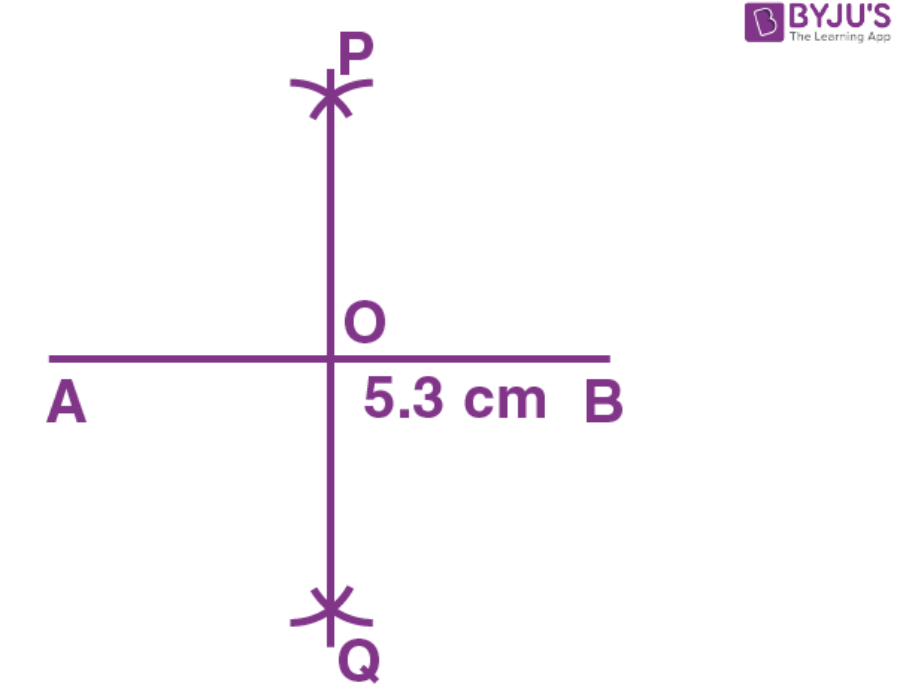1. Construct a line segment AB = 5.3cm

2. Taking A as centre and radius equal to more than half of AB, construct arcs on both sides of AB.

3. Taking B as centre and with the same radius as taken in step 2, construct arcs on both the sides of AB.

4. Let the arcs intersect each other at points P and Q.

5. Now join P and Q.

6. The line PQ cuts the given line segment AB at the point O.

Therefore, PQ is a bisector of AB such that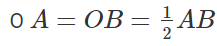Second Method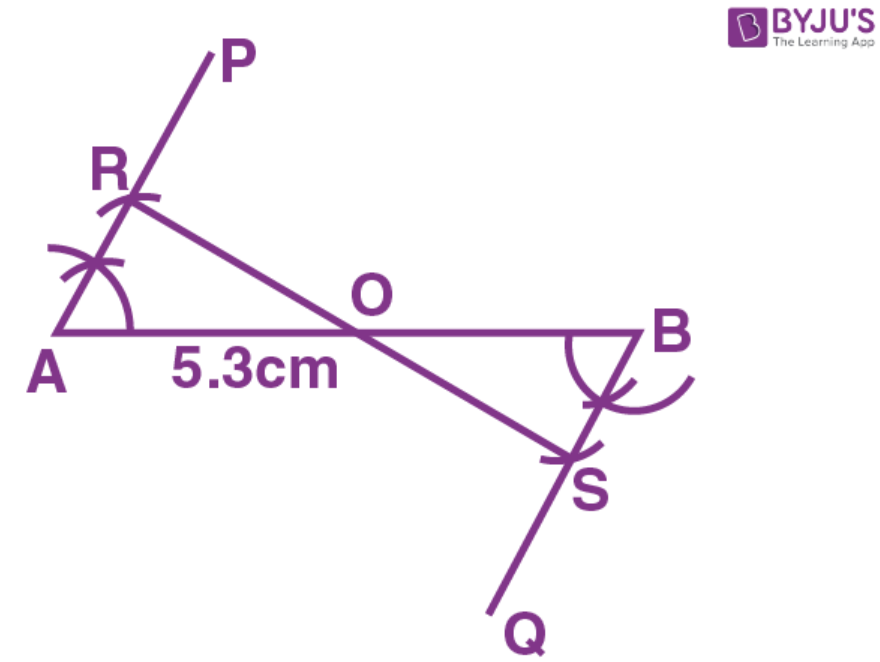Steps of Construction:

1. Construct the given line segment AB = 5.3 cm.

2. At the point A, draw ∠PAB of any suitable measure. Then ∠PAB = 60° construct ∠QBA = 60°

3. From AP, cut AR of any suitable length and from BQ; cut BS = AR.

4. Now join R and S

5. Let RS cut the given line segment AB at the point O.

Therefore, RS is a bisector of AB such that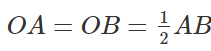Question 8.

Draw a line segment PQ = 4.8 cm. Construct the perpendicular bisector of PQ.

Solution:-

Steps of Construction: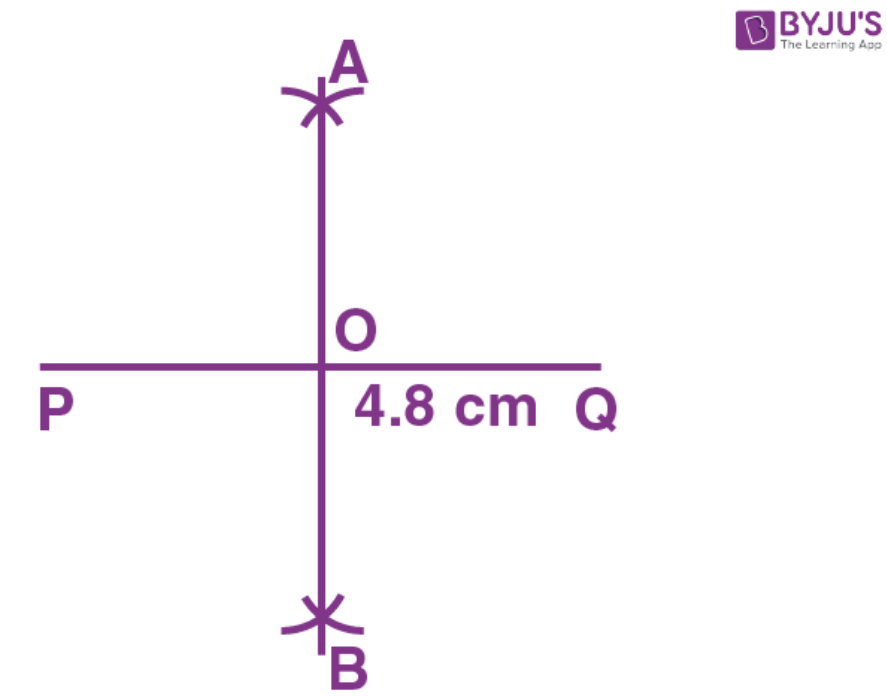1. Construct a line segment PQ = 4.8cm.

2. Taking P as centre and radius equal than half of PQ, construct arc on both the PQ.

3. Taking Q as centre and the same radius as taken in step 2, construct arcs on both sides of PQ.

4. Let the arcs intersect each other at point A and B

5. Now join A and B.

6. The line AB cuts the line segment PQ at the point O. Here OP = OQ and ∠AOQ=90° Then the line AB is perpendicular bisector of PQ.

Question 9.

In each of the following, draw perpendicular through point P to the line segment AB.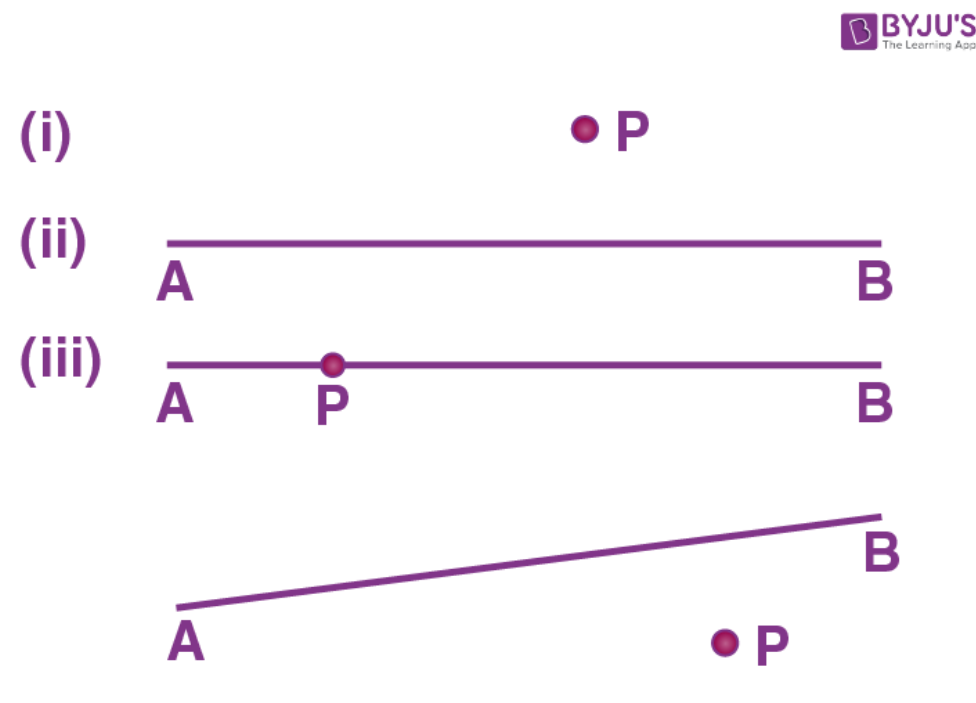Solution:-

(i) Steps of Construction: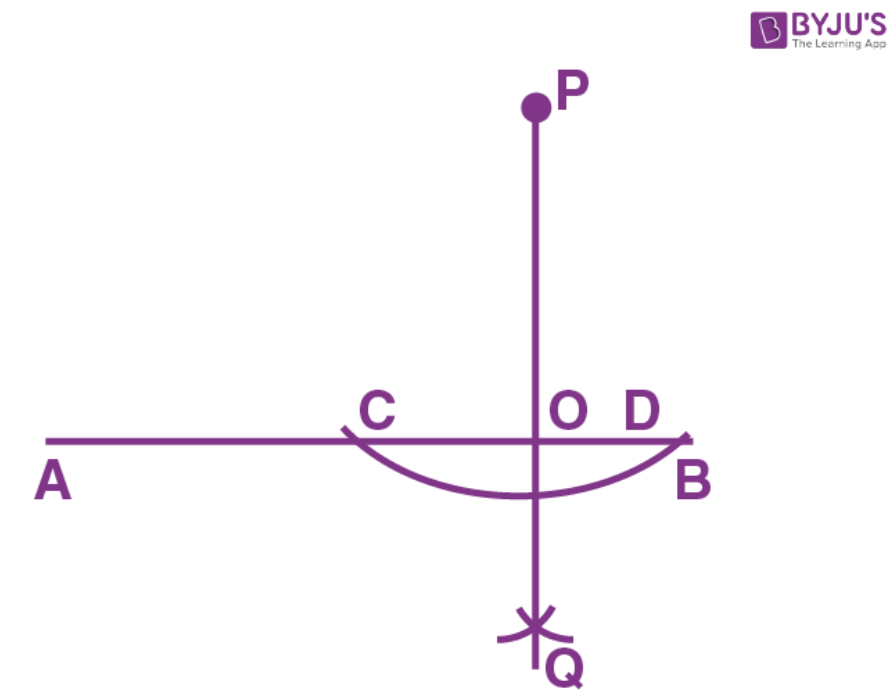1. Taking P as centre, construct an arc of a suitable radius which cuts AB at points C and D

2. Taking C and D as centers, construct arcs of equal radii and let these arcs intersect each other at the point 0 [The radius of these arcs must be more than half of CD and both the arcs must be drawn on the other side]

3. Now join P and Q

4. Let PQ cut AB at the point O.

Therefore, OP is the required perpendicular clearly, ∠AOP=∠BOP=90°

(ii) Steps of Construction: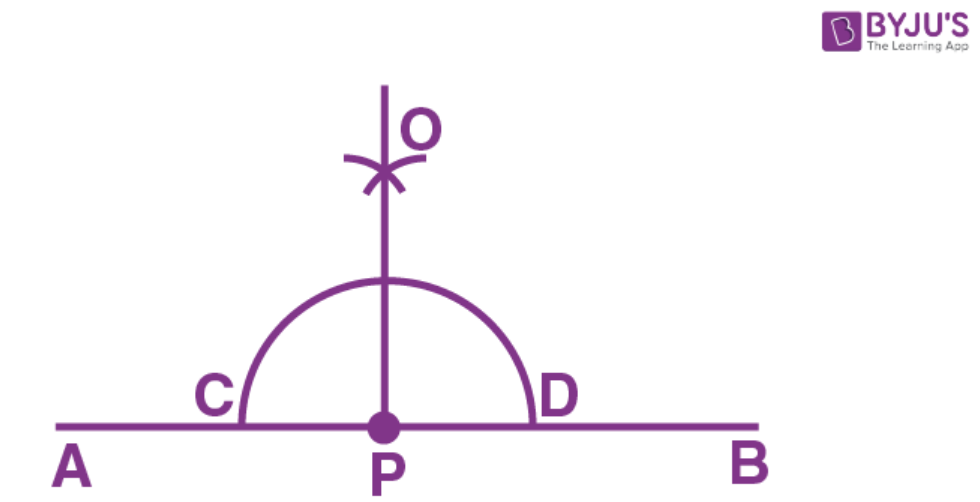1. Taking P as centre, construct an arc of any suitable radius which cuts AB at points C and D.

2. Taking C and D as centers, construct arcs of equal radii which intersect each other at point A.

[This radius must be more than half of CD and let these arc intersect each other at the point 0]

3. Now join P and O. Then OP is the required perpendicular.

∠OPA=∠OPB=90°

(iii) Steps of Construction: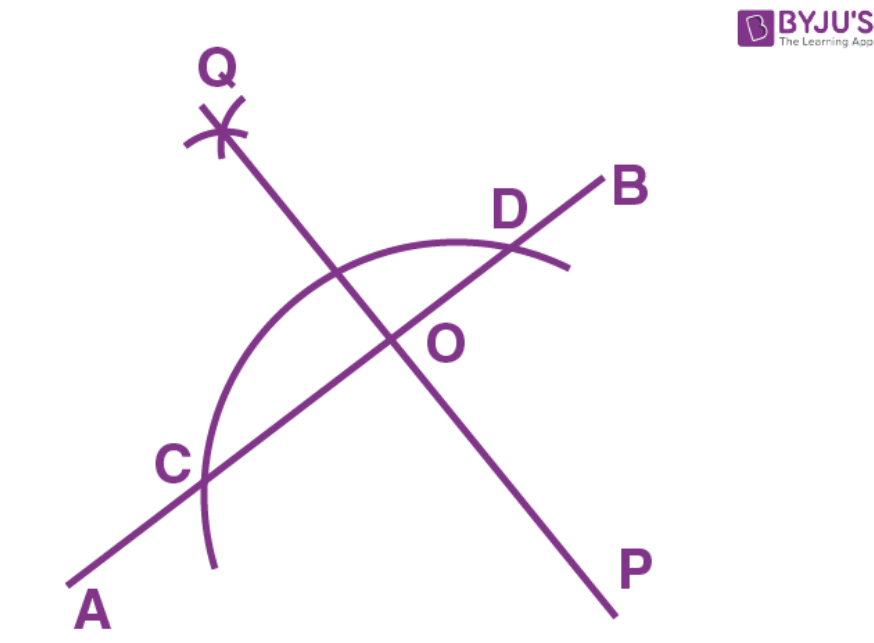1. Taking P as centre, construct an arc of any suitable radius which cuts AB at points C and D

2. Taking C and D as centre, construct arcs of equal radii

[The radius of these arcs must be more than half of CD and both the arcs must be drawn on the other side.]

And let these arcs intersect each other at the point Q.

3. Now join Q and P. Let QP cut AB at the point O. Then OP is the required perpendicular.

Clearly, ∠AOP = ∠BOP = 90°

Question 10:

Draw a line segment A B=5.5 cmMark a point P, such that P A=6 cm and P B=4.8 cm. From the point P draw perpendicular to AB.

Solution:-

Step of Construction: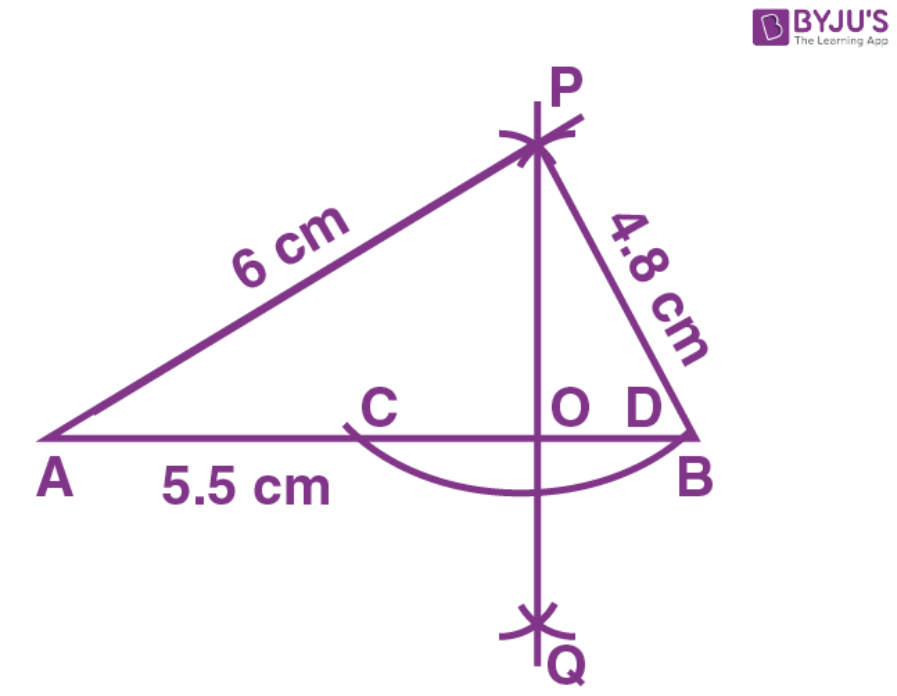1. Construct a line segment AB=5.5 cm

2. Taking A as centre and radius =6 cm construct an arc.

3. Taking B as centre and radius =4.8 cm construct another arc.

4. Let these arcs meet each other at the point P.  PA=6 cm, P B=4.8

5. Take P as centre and some suitable radius construct an arc meeting AB at the points C and D.

6. Take C as centre and radius more than half of CD construct an arc.

7. Take D as center and same radius as in step 6, construct an arc.

8. Let these arcs meet each other at the point Q.

9. Now join PQ.

10. The PQ meet AB at point O.

Then POAB i.e; ∠AOP=90° =∠POB

Question 11.

Draw a line segment AB=6.2cm. Mark a point P in AB such that BP=4cm. Through point P draw perpendicular to AB.

Solution:

Steps of Construction:

1. Construct a line segment AB=6.2cm

2. Cut off BP=4cm

3. Take P as centre and some radius construct arc meeting AB at the points C, D.

4. Take C, D as centers and equal radii [each is more than half of CD] construct two arcs, meeting each other at the point 0.

5. Now join OP. Then OP is perpendicular for AB.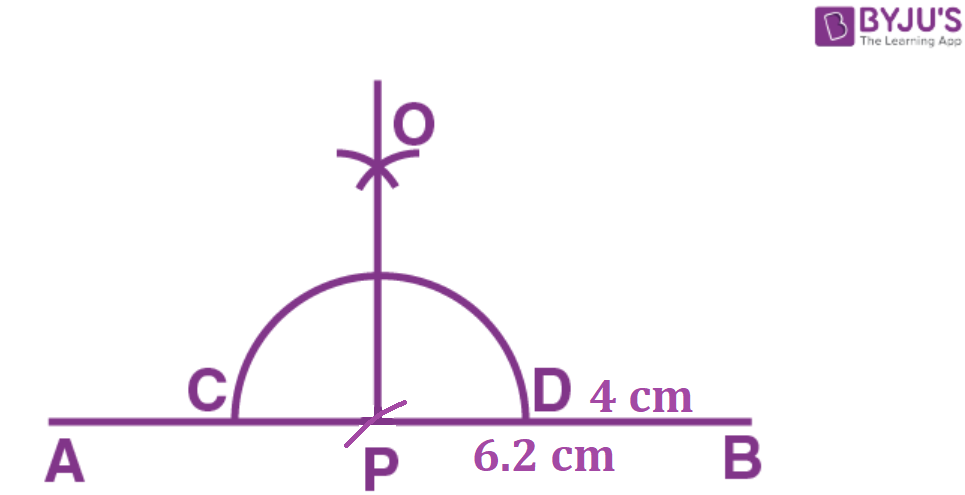Question 12.

Draw a line AB=6cm. Mark a point P anywhere outside the line AB. Through the point P, construct a line parallel to AB.

Solution:-

Steps of construction: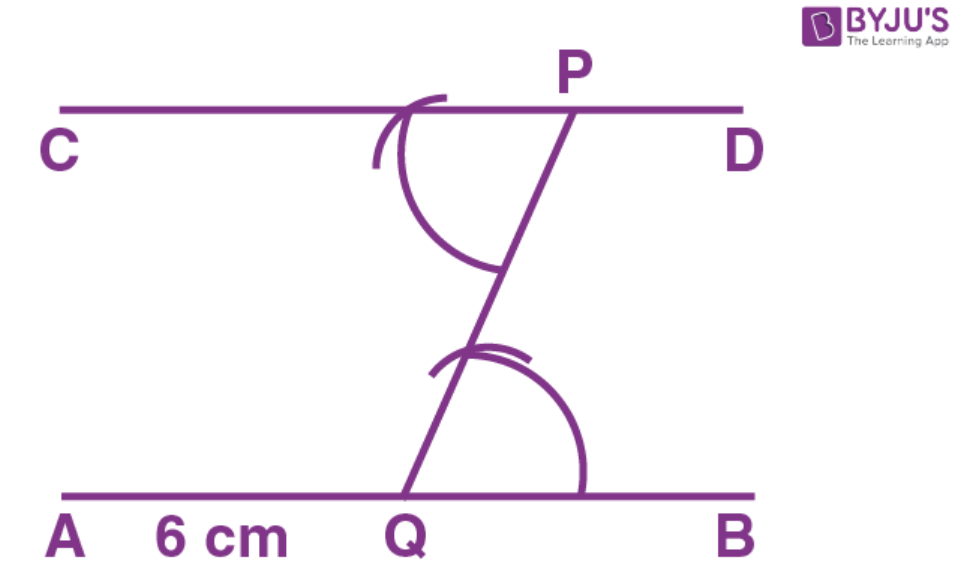1. Construct a line AB=6cm

2. Take any point Q on the line AB and join it with the given point P.

3. At point P, draw ∠CPQ=∠PQB

4. Produce CP up to any point D.

Therefore, CPD is the required parallel line.

Question 13.

Draw a line Mn=5. 8cm. Locate point A which is 4.5cm from M and 5cm from N. Through A draw a line parallel to line MN.

Solution:-

Steps of construction:

1. Construct a line MN =5.8cm

2. Taking M as centre and radius =4.5cm, construct an arc.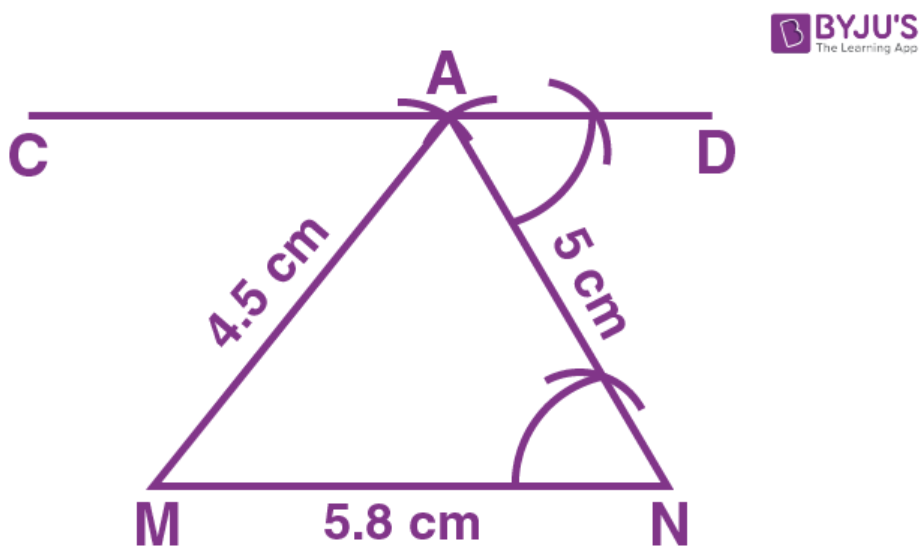3. Taking N as centre draw another arc of radius 5cm. These arcs intersect each other at A.

4. Now join AM and AN.

5. At point A, construct ∠DAN=∠ANM

6. Produce DA to any point C.

Therefore, CAD is the required parallel line.

Question 14.

Draw a straight line AB=6.5cm. Draw another line which is parallel to AB at a distance of 2.8cm from it.

Solution:-

Steps of construction: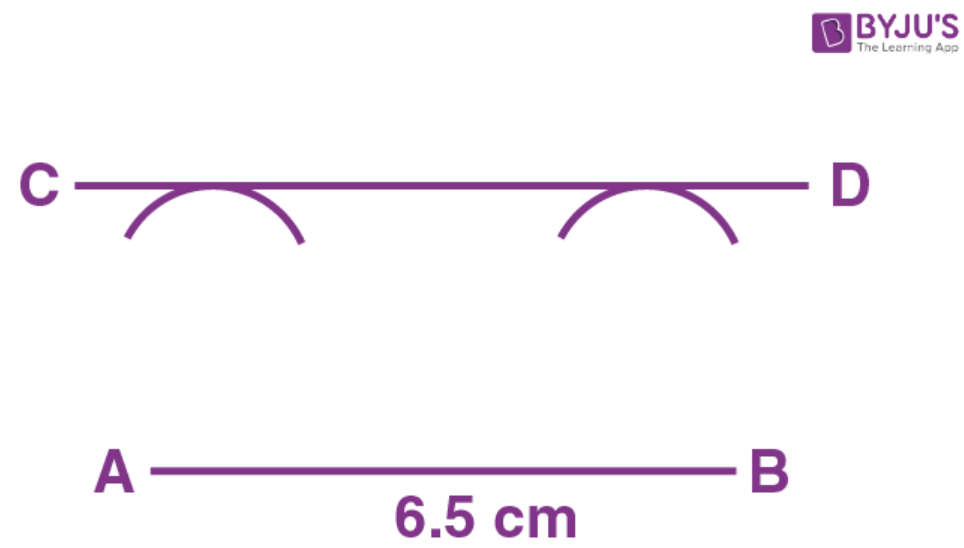1. Construct a straight line AB=6.5cm

2. With A as centre, construct an arc of radius 2.8cm.

3. With B as centre, construct another arc of radius 2.8cm.

4. Construct a line CD which touches the two arcs drawn.

Therefore, CD is the required parallel line.

Question 15.

Construct an angle PQR=80°. Draw a line parallel to PQ at a distance of 3cm from it and another line parallel to QR at a distance of 3.5cm from it. Mark the point of intersection of these parallel lines as A.

Solution:-

Steps of construction:

1. Construct ∠PQR=80°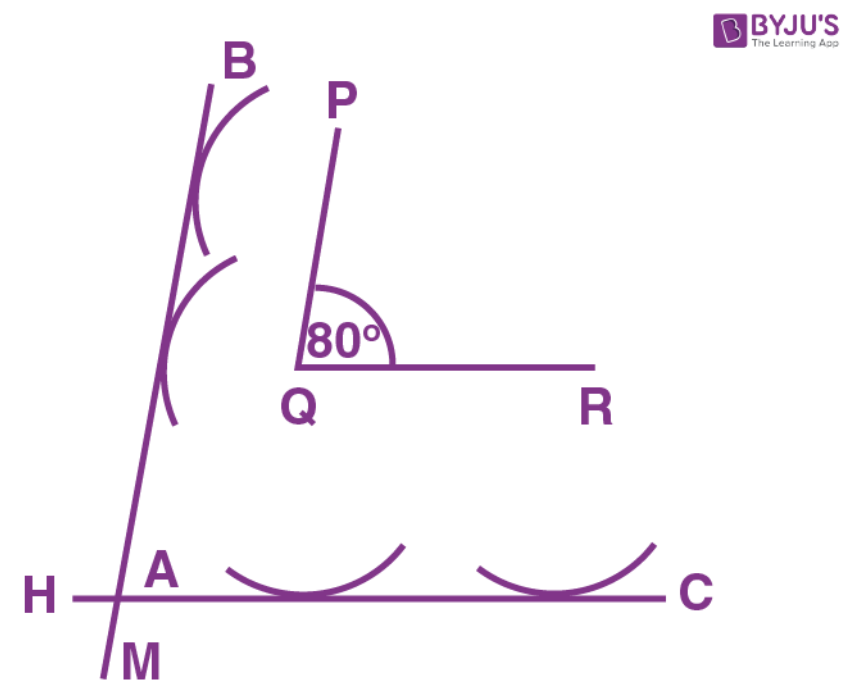2. Taking P as center construct an arc of radius 2cm.

3. Again with Q as centre, construct another arc of radius 2cm. Then BM is a line which touches the two arcs. Then BM is a line parallel to PQ.

4. Taking Q as centre, construct an arc of radius 3.5cm. Taking R as centre construct another arc of radius 3.5cm.  Construct a line HC which touches these two arcs. Let these two parallel lines intersect at A.

### ICSE Class 8 Maths Selina Solutions Chapter 18 – Constructions

Chapter 18 of ICSE Class 8 Maths provides introduction of construction of quadrilaterals and explains the steps of constructing different types of quadrilaterals. The concepts included in this chapter is explained in a simple language for better understanding of the students. All the concepts taught in this chapter will be required when you will be promoted to the next class. Moreover, students can also access ICSE Class 8 Selina Solution for other subjects like Physics, Chemistry, Biology.

Keep learning and stay tuned for further updates on ICSE and other competitive exams and subscribe to our Youtube channel.